The main idea of contrastive learning is to learn representations such that similar samples stay close to each other, while dissimilar ones are far apart. Contrastive learning can be applied to both supervised and unsupervised data and has been shown to achieve good performance on a variety of vision and language tasks.

The goal of contrastive representation learning is to learn such an embedding space in which similar sample pairs stay close to each other while dissimilar ones are far apart. Contrastive learning can be applied to both supervised and unsupervised settings. When working with unsupervised data, contrastive learning is one of the most powerful approaches in self-supervised learning.

## Contrastive Training Objectives

In early versions of loss functions for contrastive learning, only one positive and one negative sample are involved. The trend in recent training objectives is to include multiple positive and negative pairs in one batch.

### Contrastive Loss

Contrastive loss (Chopra et al. 2005) is one of the earliest training objectives used for deep metric learning in a contrastive fashion.

Given a list of input samples $$\{ \mathbf{x}_i \}$$, each has a corresponding label $$y_i \in \{1, \dots, L\}$$ among $$L$$ classes. We would like to learn a function $$f_\theta(.): \mathcal{X}\to\mathbb{R}^d$$ that encodes $$x_i$$ into an embedding vector such that examples from the same class have similar embeddings and samples from different classes have very different ones. Thus, contrastive loss takes a pair of inputs $$(x_i, x_j)$$ and minimizes the embedding distance when they are from the same class but maximizes the distance otherwise.

$\mathcal{L}_\text{cont}(\mathbf{x}_i, \mathbf{x}_j, \theta) = \mathbb{1}[y_i=y_j] \| f_\theta(\mathbf{x}_i) - f_\theta(\mathbf{x}_j) \|^2_2 + \mathbb{1}[y_i\neq y_j]\max(0, \epsilon - \|f_\theta(\mathbf{x}_i) - f_\theta(\mathbf{x}_j)\|_2)^2$

where $$\epsilon$$ is a hyperparameter, defining the lower bound distance between samples of different classes.

### Triplet Loss

Triplet loss was originally proposed in the FaceNet (Schroff et al. 2015) paper and was used to learn face recognition of the same person at different poses and angles.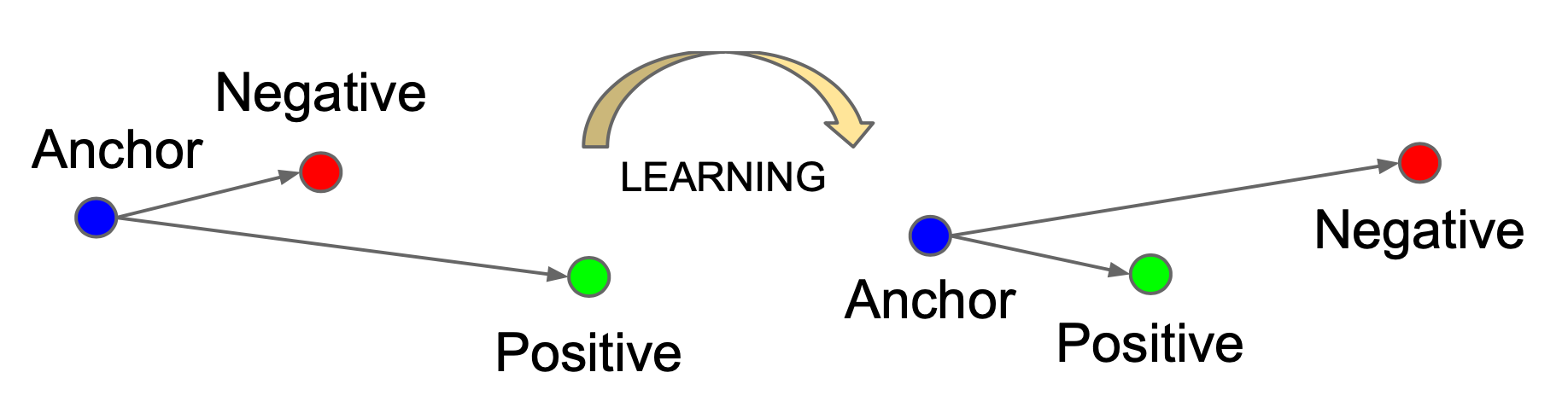Fig. 1. Illustration of triplet loss given one positive and one negative per anchor. (Image source: Schroff et al. 2015)

Given one anchor input $$\mathbf{x}$$, we select one positive sample $$\mathbf{x}^+$$ and one negative $$\mathbf{x}^-$$, meaning that $$\mathbf{x}^+$$ and $$\mathbf{x}$$ belong to the same class and $$\mathbf{x}^-$$ is sampled from another different class. Triplet loss learns to minimize the distance between the anchor $$\mathbf{x}$$ and positive $$\mathbf{x}^+$$ and maximize the distance between the anchor $$\mathbf{x}$$ and negative $$\mathbf{x}^-$$ at the same time with the following equation:

$\mathcal{L}_\text{triplet}(\mathbf{x}, \mathbf{x}^+, \mathbf{x}^-) = \sum_{\mathbf{x} \in \mathcal{X}} \max\big( 0, \|f(\mathbf{x}) - f(\mathbf{x}^+)\|^2_2 - \|f(\mathbf{x}) - f(\mathbf{x}^-)\|^2_2 + \epsilon \big)$

where the margin parameter $$\epsilon$$ is configured as the minimum offset between distances of similar vs dissimilar pairs.

It is crucial to select challenging $$\mathbf{x}^-$$ to truly improve the model.

### Lifted Structured Loss

Lifted Structured Loss (Song et al. 2015) utilizes all the pairwise edges within one training batch for better computational efficiency.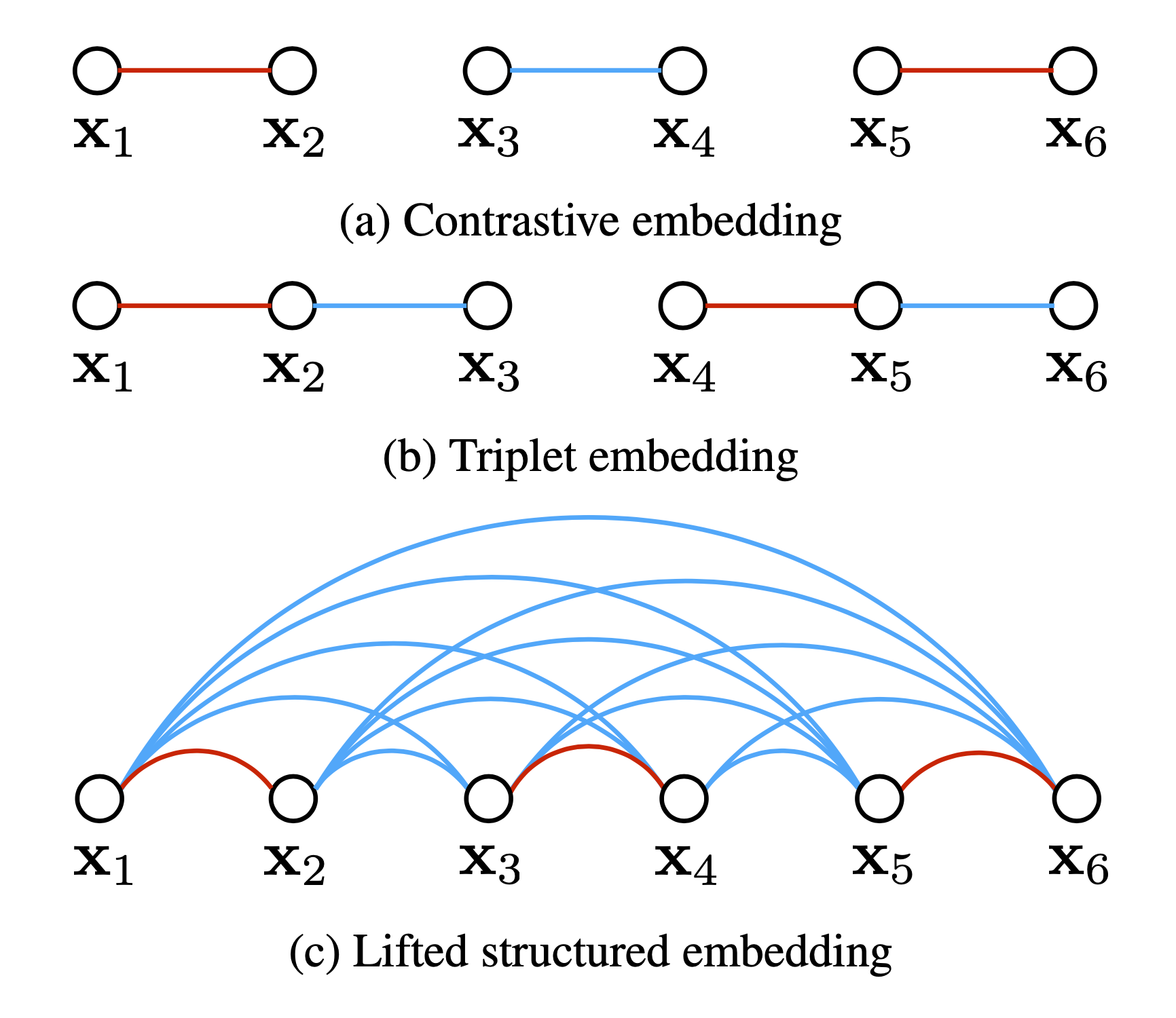Fig. 2. Illustration compares contrastive loss, triplet loss and lifted structured loss. Red and blue edges connect similar and dissimilar sample pairs respectively. (Image source: Song et al. 2015)

Let $$D_{ij} = \| f(\mathbf{x}_i) - f(\mathbf{x}_j) \|_2$$, a structured loss function is defined as

\begin{aligned} \mathcal{L}_\text{struct} &= \frac{1}{2\vert \mathcal{P} \vert} \sum_{(i,j) \in \mathcal{P}} \max(0, \mathcal{L}_\text{struct}^{(ij)})^2 \\ \text{where } \mathcal{L}_\text{struct}^{(ij)} &= D_{ij} + \color{red}{\max \big( \max_{(i,k)\in \mathcal{N}} \epsilon - D_{ik}, \max_{(j,l)\in \mathcal{N}} \epsilon - D_{jl} \big)} \end{aligned}

where $$\mathcal{P}$$ contains the set of positive pairs and $$\mathcal{N}$$ is the set of negative pairs. Note that the dense pairwise squared distance matrix can be easily computed per training batch.

The red part in $$\mathcal{L}_\text{struct}^{(ij)}$$ is used for mining hard negatives. However, it is not smooth and may cause the convergence to a bad local optimum in practice. Thus, it is relaxed to be:

$\mathcal{L}_\text{struct}^{(ij)} = D_{ij} + \log \Big( \sum_{(i,k)\in\mathcal{N}} \exp(\epsilon - D_{ik}) + \sum_{(j,l)\in\mathcal{N}} \exp(\epsilon - D_{jl}) \Big)$

In the paper, they also proposed to enhance the quality of negative samples in each batch by actively incorporating difficult negative samples given a few random positive pairs.

### N-pair Loss

Multi-Class N-pair loss (Sohn 2016) generalizes triplet loss to include comparison with multiple negative samples.

Given a $$(N + 1)$$-tuplet of training samples, $$\{ \mathbf{x}, \mathbf{x}^+, \mathbf{x}^-_1, \dots, \mathbf{x}^-_{N-1} \}$$, including one positive and $$N-1$$ negative ones, N-pair loss is defined as:

\begin{aligned} \mathcal{L}_\text{N-pair}(\mathbf{x}, \mathbf{x}^+, \{\mathbf{x}^-_i\}^{N-1}_{i=1}) &= \log\big(1 + \sum_{i=1}^{N-1} \exp(f(\mathbf{x})^\top f(\mathbf{x}^-_i) - f(\mathbf{x})^\top f(\mathbf{x}^+))\big) \\ &= -\log\frac{\exp(f(\mathbf{x})^\top f(\mathbf{x}^+))}{\exp(f(\mathbf{x})^\top f(\mathbf{x}^+)) + \sum_{i=1}^{N-1} \exp(f(\mathbf{x})^\top f(\mathbf{x}^-_i))} \end{aligned}

If we only sample one negative sample per class, it is equivalent to the softmax loss for multi-class classification.

### NCE

Noise Contrastive Estimation, short for NCE, is a method for estimating parameters of a statistical model, proposed by Gutmann & Hyvarinen in 2010. The idea is to run logistic regression to tell apart the target data from noise. Read more on how NCE is used for learning word embedding here.

Let $$\mathbf{x}$$ be the target sample $$\sim P(\mathbf{x} \vert C=1; \theta) = p_\theta(\mathbf{x})$$ and $$\tilde{\mathbf{x}}$$ be the noise sample $$\sim P(\tilde{\mathbf{x}} \vert C=0) = q(\tilde{\mathbf{x}})$$. Note that the logistic regression models the logit (i.e. log-odds) and in this case we would like to model the logit of a sample $$u$$ from the target data distribution instead of the noise distribution:

$\ell_\theta(\mathbf{u}) = \log \frac{p_\theta(\mathbf{u})}{q(\mathbf{u})} = \log p_\theta(\mathbf{u}) - \log q(\mathbf{u})$

After converting logits into probabilities with sigmoid $$\sigma(.)$$, we can apply cross entropy loss:

\begin{aligned} \mathcal{L}_\text{NCE} &= - \frac{1}{N} \sum_{i=1}^N \big[ \log \sigma (\ell_\theta(\mathbf{x}_i)) + \log (1 - \sigma (\ell_\theta(\tilde{\mathbf{x}}_i))) \big] \\ \text{ where }\sigma(\ell) &= \frac{1}{1 + \exp(-\ell)} = \frac{p_\theta}{p_\theta + q} \end{aligned}

Here I listed the original form of NCE loss which works with only one positive and one noise sample. In many follow-up works, contrastive loss incorporating multiple negative samples is also broadly referred to as NCE.

### InfoNCE

The InfoNCE loss in CPC (Contrastive Predictive Coding; van den Oord, et al. 2018), inspired by NCE, uses categorical cross-entropy loss to identify the positive sample amongst a set of unrelated noise samples.

Given a context vector $$\mathbf{c}$$, the positive sample should be drawn from the conditional distribution $$p(\mathbf{x} \vert \mathbf{c})$$, while $$N-1$$ negative samples are drawn from the proposal distribution $$p(\mathbf{x})$$, independent from the context $$\mathbf{c}$$. For brevity, let us label all the samples as $$X=\{ \mathbf{x}_i \}^N_{i=1}$$ among which only one of them $$\mathbf{x}_\texttt{pos}$$ is a positive sample. The probability of we detecting the positive sample correctly is:

$p(C=\texttt{pos} \vert X, \mathbf{c}) = \frac{p(x_\texttt{pos} \vert \mathbf{c}) \prod_{i=1,\dots,N; i \neq \texttt{pos}} p(\mathbf{x}_i)}{\sum_{j=1}^N \big[ p(\mathbf{x}_j \vert \mathbf{c}) \prod_{i=1,\dots,N; i \neq j} p(\mathbf{x}_i) \big]} = \frac{ \frac{p(\mathbf{x}_\texttt{pos}\vert c)}{p(\mathbf{x}_\texttt{pos})} }{ \sum_{j=1}^N \frac{p(\mathbf{x}_j\vert \mathbf{c})}{p(\mathbf{x}_j)} } = \frac{f(\mathbf{x}_\texttt{pos}, \mathbf{c})}{ \sum_{j=1}^N f(\mathbf{x}_j, \mathbf{c}) }$

where the scoring function is $$f(\mathbf{x}, \mathbf{c}) \propto \frac{p(\mathbf{x}\vert\mathbf{c})}{p(\mathbf{x})}$$.

The InfoNCE loss optimizes the negative log probability of classifying the positive sample correctly:

$\mathcal{L}_\text{InfoNCE} = - \mathbb{E} \Big[\log \frac{f(\mathbf{x}, \mathbf{c})}{\sum_{\mathbf{x}' \in X} f(\mathbf{x}', \mathbf{c})} \Big]$

The fact that $$f(x, c)$$ estimates the density ratio $$\frac{p(x\vert c)}{p(x)}$$ has a connection with mutual information optimization. To maximize the the mutual information between input $$x$$ and context vector $$c$$, we have:

$I(\mathbf{x}; \mathbf{c}) = \sum_{\mathbf{x}, \mathbf{c}} p(\mathbf{x}, \mathbf{c}) \log\frac{p(\mathbf{x}, \mathbf{c})}{p(\mathbf{x})p(\mathbf{c})} = \sum_{\mathbf{x}, \mathbf{c}} p(\mathbf{x}, \mathbf{c})\log\color{blue}{\frac{p(\mathbf{x}|\mathbf{c})}{p(\mathbf{x})}}$

where the logarithmic term in blue is estimated by $$f$$.

For sequence prediction tasks, rather than modeling the future observations $$p_k(\mathbf{x}_{t+k} \vert \mathbf{c}_t)$$ directly (which could be fairly expensive), CPC models a density function to preserve the mutual information between $$\mathbf{x}_{t+k}$$ and $$\mathbf{c}_t$$:

$f_k(\mathbf{x}_{t+k}, \mathbf{c}_t) = \exp(\mathbf{z}_{t+k}^\top \mathbf{W}_k \mathbf{c}_t) \propto \frac{p(\mathbf{x}_{t+k}\vert\mathbf{c}_t)}{p(\mathbf{x}_{t+k})}$

where $$\mathbf{z}_{t+k}$$ is the encoded input and $$\mathbf{W}_k$$ is a trainable weight matrix.

### Soft-Nearest Neighbors Loss

Soft-Nearest Neighbors Loss (Salakhutdinov & Hinton 2007, Frosst et al. 2019) extends it to include multiple positive samples.

Given a batch of samples, $$\{\mathbf{x}_i, y_i)\}^B_{i=1}$$ where $$y_i$$ is the class label of $$\mathbf{x}_i$$ and a function $$f(.,.)$$ for measuring similarity between two inputs, the soft nearest neighbor loss at temperature $$\tau$$ is defined as:

$\mathcal{L}_\text{snn} = -\frac{1}{B}\sum_{i=1}^B \log \frac{\sum_{i\neq j, y_i = y_j, j=1,\dots,B} \exp(- f(\mathbf{x}_i, \mathbf{x}_j) / \tau)}{\sum_{i\neq k, k=1,\dots,B} \exp(- f(\mathbf{x}_i, \mathbf{x}_k) /\tau)}$

The temperature $$\tau$$ is used for tuning how concentrated the features are in the representation space. For example, when at low temperature, the loss is dominated by the small distances and widely separated representations cannot contribute much and become irrelevant.

### Common Setup

We can loosen the definition of “classes” and “labels” in soft nearest-neighbor loss to create positive and negative sample pairs out of unsupervised data by, for example, applying data augmentation to create noise versions of original samples.

Most recent studies follow the following definition of contrastive learning objective to incorporate multiple positive and negative samples. According to the setup in (Wang & Isola 2020), let $$p_\texttt{data}(.)$$ be the data distribution over $$\mathbb{R}^n$$ and $$p_\texttt{pos}(., .)$$ be the distribution of positive pairs over $$\mathbb{R}^{n \times n}$$. These two distributions should satisfy:

• Symmetry: $$\forall \mathbf{x}, \mathbf{x}^+, p_\texttt{pos}(\mathbf{x}, \mathbf{x}^+) = p_\texttt{pos}(\mathbf{x}^+, \mathbf{x})$$
• Matching marginal: $$\forall \mathbf{x}, \int p_\texttt{pos}(\mathbf{x}, \mathbf{x}^+) d\mathbf{x}^+ = p_\texttt{data}(\mathbf{x})$$

To learn an encoder $$f(\mathbf{x})$$ to learn a L2-normalized feature vector, the contrastive learning objective is:

\begin{aligned} \mathcal{L}_\text{contrastive} &= \mathbb{E}_{(\mathbf{x},\mathbf{x}^+)\sim p_\texttt{pos}, \{\mathbf{x}^-_i\}^M_{i=1} \overset{\text{i.i.d}}{\sim} p_\texttt{data} } \Big[ -\log\frac{\exp(f(\mathbf{x})^\top f(\mathbf{x}^+) / \tau)}{ \exp(f(\mathbf{x})^\top f(\mathbf{x}^+) / \tau) + \sum_{i=1}^M \exp(f(\mathbf{x})^\top f(\mathbf{x}_i^-) / \tau)} \Big] & \\ &\approx \mathbb{E}_{(\mathbf{x},\mathbf{x}^+)\sim p_\texttt{pos}, \{\mathbf{x}^-_i\}^M_{i=1} \overset{\text{i.i.d}}{\sim} p_\texttt{data} }\Big[ - f(\mathbf{x})^\top f(\mathbf{x}^+) / \tau + \log\big(\sum_{i=1}^M \exp(f(\mathbf{x})^\top f(\mathbf{x}_i^-) / \tau)\big) \Big] & \scriptstyle{\text{; Assuming infinite negatives}} \\ &= -\frac{1}{\tau}\mathbb{E}_{(\mathbf{x},\mathbf{x}^+)\sim p_\texttt{pos}}f(\mathbf{x})^\top f(\mathbf{x}^+) + \mathbb{E}_{ \mathbf{x} \sim p_\texttt{data}} \Big[ \log \mathbb{E}_{\mathbf{x}^- \sim p_\texttt{data}} \big[ \sum_{i=1}^M \exp(f(\mathbf{x})^\top f(\mathbf{x}_i^-) / \tau)\big] \Big] & \end{aligned}

## Key Ingredients

### Heavy Data Augmentation

Given a training sample, data augmentation techniques are needed for creating noise versions of itself to feed into the loss as positive samples. Proper data augmentation setup is critical for learning good and generalizable embedding features. It introduces the non-essential variations into examples without modifying semantic meanings and thus encourages the model to learn the essential part of the representation. For example, experiments in SimCLR showed that the composition of random cropping and random color distortion is crucial for good performance on learning visual representation of images.

### Large Batch Size

Using a large batch size during training is another key ingredient in the success of many contrastive learning methods (e.g. SimCLR, CLIP), especially when it relies on in-batch negatives. Only when the batch size is big enough, the loss function can cover a diverse enough collection of negative samples, challenging enough for the model to learn meaningful representation to distinguish different examples.

### Hard Negative Mining

Hard negative samples should have different labels from the anchor sample, but have embedding features very close to the anchor embedding. With access to ground truth labels in supervised datasets, it is easy to identify task-specific hard negatives. For example when learning sentence embedding, we can treat sentence pairs labelled as “contradiction” in NLI datasets as hard negative pairs (e.g. SimCSE, or use top incorrect candidates returned by BM25 with most keywords matched as hard negative samples (DPR; Karpukhin et al., 2020).

However, it becomes tricky to do hard negative mining when we want to remain unsupervised. Increasing training batch size or memory bank size implicitly introduces more hard negative samples, but it leads to a heavy burden of large memory usage as a side effect.

Chuang et al. (2020) studied the sampling bias in contrastive learning and proposed debiased loss. In the unsupervised setting, since we do not know the ground truth labels, we may accidentally sample false negative samples. Sampling bias can lead to significant performance drop.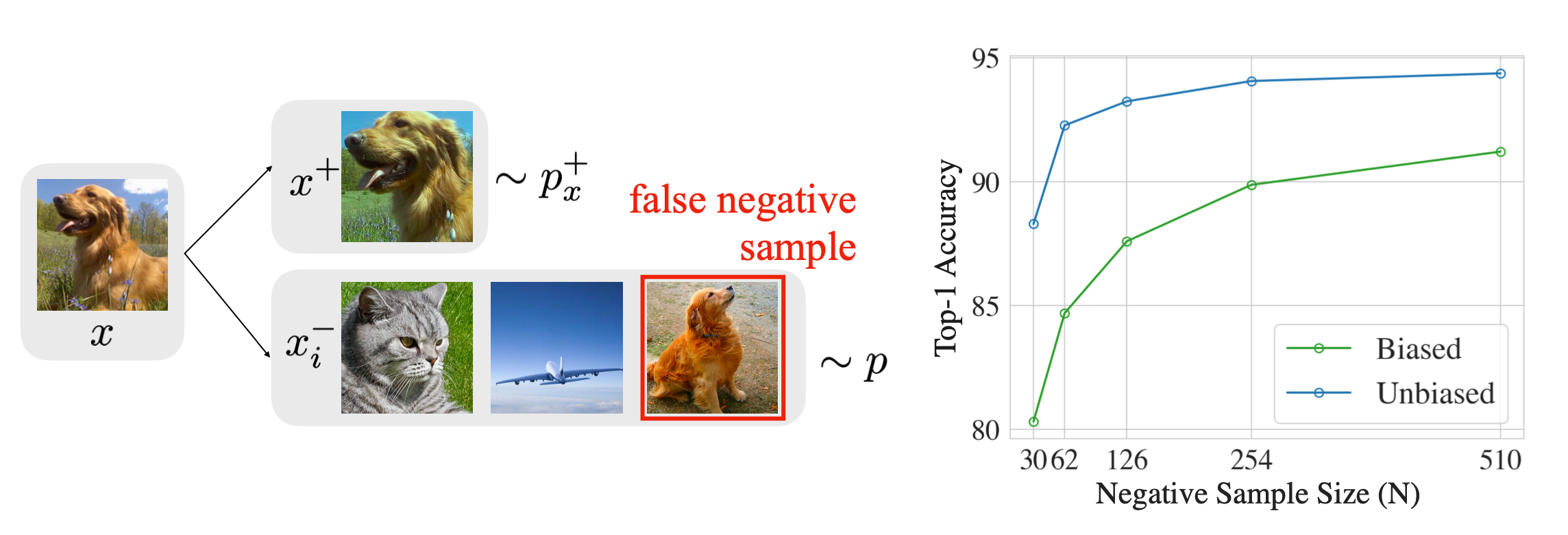Fig. 3. Sampling bias which refers to false negative samples in contrastive learning can lead to a big performance drop. (Image source: Chuang et al., 2020)

Let us assume the probability of anchor class $$c$$ is uniform $$\rho(c)=\eta^+$$ and the probability of observing a different class is $$\eta^- = 1-\eta^+$$.

• The probability of observing a positive example for $$\mathbf{x}$$ is $$p^+_x(\mathbf{x}')=p(\mathbf{x}'\vert \mathbf{h}_{x'}=\mathbf{h}_x)$$;
• The probability of getting a negative sample for $$\mathbf{x}$$ is $$p^-_x(\mathbf{x}')=p(\mathbf{x}'\vert \mathbf{h}_{x'}\neq\mathbf{h}_x)$$.

When we are sampling $$\mathbf{x}^-$$ , we cannot access the true $$p^-_x(\mathbf{x}^-)$$ and thus $$\mathbf{x}^-$$ may be sampled from the (undesired) anchor class $$c$$ with probability $$\eta^+$$. The actual sampling data distribution becomes:

$p(\mathbf{x}') = \eta^+ p^+_x(\mathbf{x}') + \eta^- p_x^-(\mathbf{x}')$

Thus we can use $$p^-_x(\mathbf{x}') = (p(\mathbf{x}') - \eta^+ p^+_x(\mathbf{x}'))/\eta^-$$ for sampling $$\mathbf{x}^-$$ to debias the loss. With $$N$$ samples $$\{\mathbf{u}_i\}^N_{i=1}$$ from $$p$$ and $$M$$ samples $$\{ \mathbf{v}_i \}_{i=1}^M$$ from $$p^+_x$$ , we can estimate the expectation of the second term $$\mathbb{E}_{\mathbf{x}^-\sim p^-_x}[\exp(f(\mathbf{x})^\top f(\mathbf{x}^-))]$$ in the denominator of contrastive learning loss:

$g(\mathbf{x}, \{\mathbf{u}_i\}^N_{i=1}, \{\mathbf{v}_i\}_{i=1}^M) = \max\Big\{ \frac{1}{\eta^-}\Big( \frac{1}{N}\sum_{i=1}^N \exp(f(\mathbf{x})^\top f(\mathbf{u}_i)) - \frac{\eta^+}{M}\sum_{i=1}^M \exp(f(\mathbf{x})^\top f(\mathbf{v}_i)) \Big), \exp(-1/\tau) \Big\}$

where $$\tau$$ is the temperature and $$\exp(-1/\tau)$$ is the theoretical lower bound of $$\mathbb{E}_{\mathbf{x}^-\sim p^-_x}[\exp(f(\mathbf{x})^\top f(\mathbf{x}^-))]$$.

The final debiased contrastive loss looks like:

$\mathcal{L}^{N,M}_\text{debias}(f) = \mathbb{E}_{\mathbf{x},\{\mathbf{u}_i\}^N_{i=1}\sim p;\;\mathbf{x}^+, \{\mathbf{v}_i\}_{i=1}^M\sim p^+} \Big[ -\log\frac{\exp(f(\mathbf{x})^\top f(\mathbf{x}^+)}{\exp(f(\mathbf{x})^\top f(\mathbf{x}^+) + N g(x,\{\mathbf{u}_i\}^N_{i=1}, \{\mathbf{v}_i\}_{i=1}^M)} \Big]$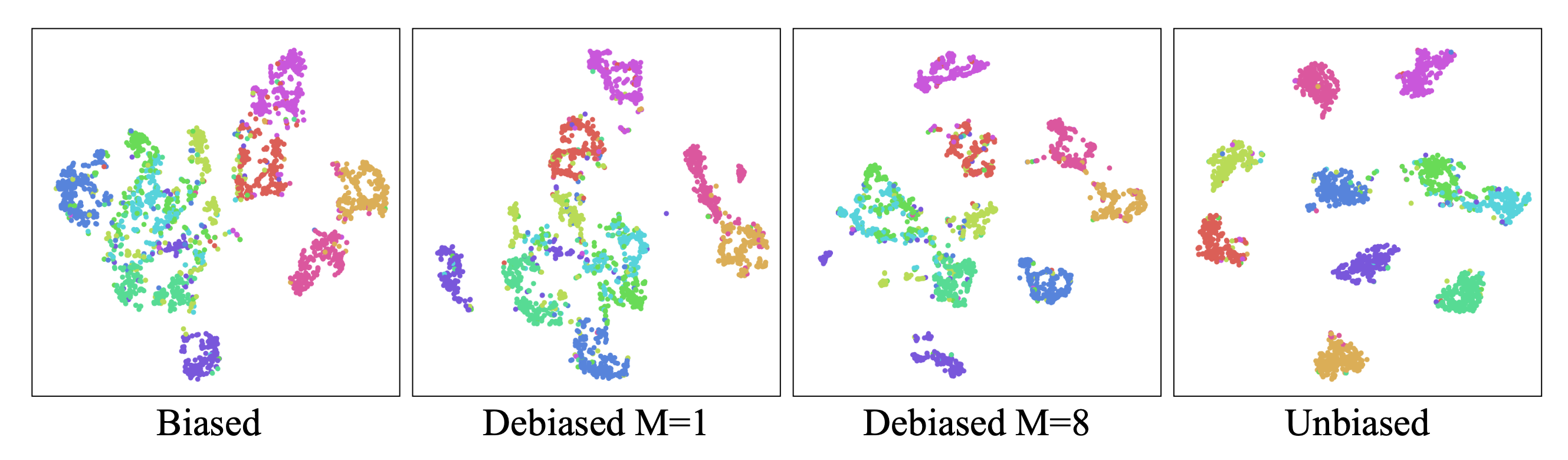Fig. 4. t-SNE visualization of learned representation with debiased contrastive learning. (Image source: Chuang et al., 2020)

Following the above annotation, Robinson et al. (2021) modified the sampling probabilities to target at hard negatives by up-weighting the probability $$p^-_x(x')$$ to be proportional to its similarity to the anchor sample. The new sampling probability $$q_\beta(x^-)$$ is:

$q_\beta(\mathbf{x}^-) \propto \exp(\beta f(\mathbf{x})^\top f(\mathbf{x}^-)) \cdot p(\mathbf{x}^-)$

where $$\beta$$ is a hyperparameter to tune.

We can estimate the second term in the denominator $$\mathbb{E}_{\mathbf{x}^- \sim q_\beta} [\exp(f(\mathbf{x})^\top f(\mathbf{x}^-))]$$ using importance sampling where both the partition functions $$Z_\beta, Z^+_\beta$$ can be estimated empirically.

\begin{aligned} \mathbb{E}_{\mathbf{u} \sim q_\beta} [\exp(f(\mathbf{x})^\top f(\mathbf{u}))] &= \mathbb{E}_{\mathbf{u} \sim p} [\frac{q_\beta}{p}\exp(f(\mathbf{x})^\top f(\mathbf{u}))] = \mathbb{E}_{\mathbf{u} \sim p} [\frac{1}{Z_\beta}\exp((\beta + 1)f(\mathbf{x})^\top f(\mathbf{u}))] \\ \mathbb{E}_{\mathbf{v} \sim q^+_\beta} [\exp(f(\mathbf{x})^\top f(\mathbf{v}))] &= \mathbb{E}_{\mathbf{v} \sim p^+} [\frac{q^+_\beta}{p}\exp(f(\mathbf{x})^\top f(\mathbf{v}))] = \mathbb{E}_{\mathbf{v} \sim p} [\frac{1}{Z^+_\beta}\exp((\beta + 1)f(\mathbf{x})^\top f(\mathbf{v}))] \end{aligned}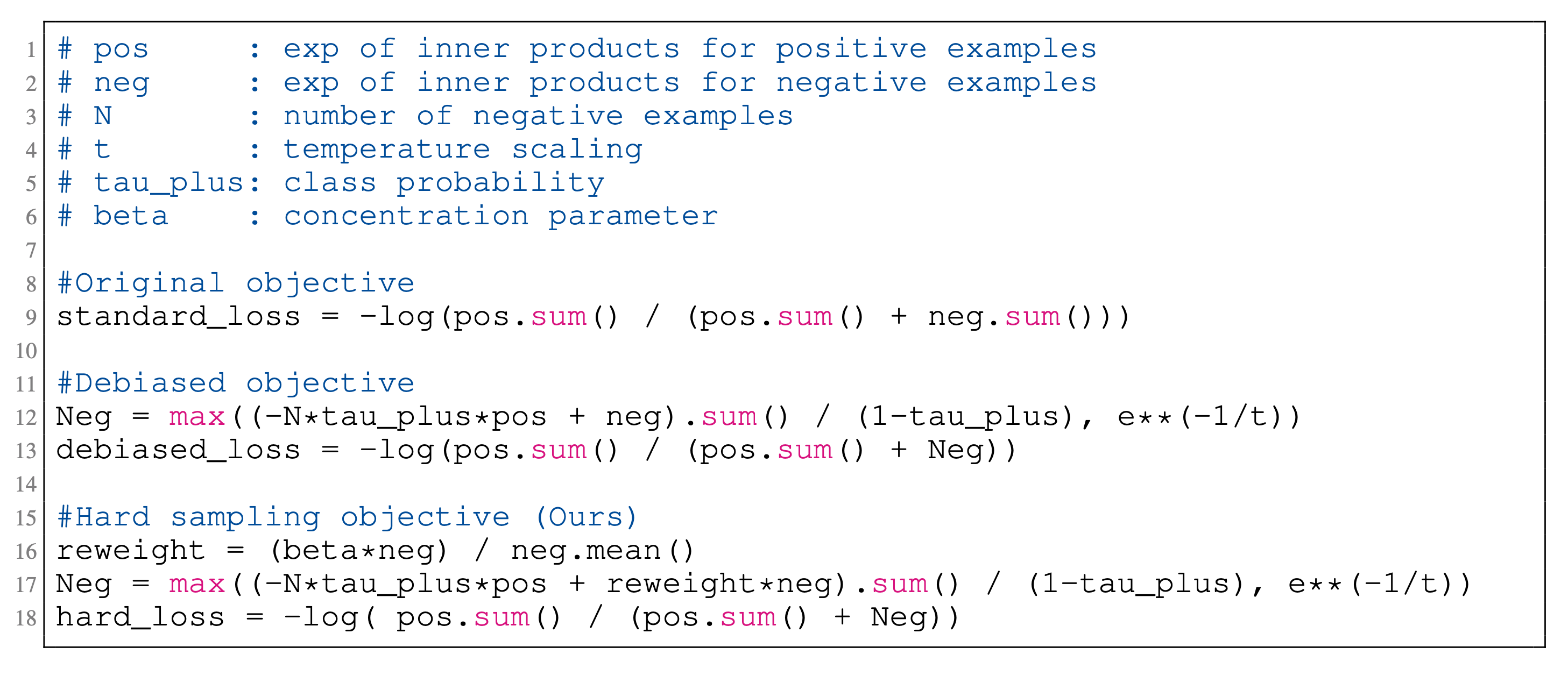Fig. 5. Pseudo code for computing NCE loss, debiased contrastive loss, and hard negative sample objective when setting $$M=1$$. (Image source: Robinson et al., 2021 )

## Vision: Image Embedding

### Image Augmentations

Most approaches for contrastive representation learning in the vision domain rely on creating a noise version of a sample by applying a sequence of data augmentation techniques. The augmentation should significantly change its visual appearance but keep the semantic meaning unchanged.

#### Basic Image Augmentation

There are many ways to modify an image while retaining its semantic meaning. We can use any one of the following augmentation or a composition of multiple operations.

• Random cropping and then resize back to the original size.
• Random color distortions
• Random Gaussian blur
• Random color jittering
• Random horizontal flip
• Random grayscale conversion
• Multi-crop augmentation: Use two standard resolution crops and sample a set of additional low resolution crops that cover only small parts of the image. Using low resolution crops reduces the compute cost. (SwAV)
• And many more …

#### Augmentation Strategies

Many frameworks are designed for learning good data augmentation strategies (i.e. a composition of multiple transforms). Here are a few common ones.

• AutoAugment (Cubuk, et al. 2018): Inspired by NAS, AutoAugment frames the problem of learning best data augmentation operations (i.e. shearing, rotation, invert, etc.) for image classification as an RL problem and looks for the combination that leads to the highest accuracy on the evaluation set.
• RandAugment (Cubuk et al., 2019): RandAugment greatly reduces the search space of AutoAugment by controlling the magnitudes of different transformation operations with a single magnitude parameter.
• PBA (Population based augmentation; Ho et al., 2019): PBA combined PBT (Jaderberg et al, 2017) with AutoAugment, using the evolutionary algorithm to train a population of children models in parallel to evolve the best augmentation strategies.
• UDA (Unsupervised Data Augmentation; Xie et al., 2019): Among a set of possible augmentation strategies, UDA selects those to minimize the KL divergence between the predicted distribution over an unlabelled example and its unlabelled augmented version.

#### Image Mixture

Image mixture methods can construct new training examples from existing data points.

• Mixup (Zhang et al., 2018): It runs global-level mixture by creating a weighted pixel-wise combination of two existing images $$I_1$$ and $$I_2$$: $$I_\text{mixup} \gets \alpha I_1 + (1-\alpha) I_2$$ and $$\alpha \in [0, 1]$$.
• Cutmix (Yun et al., 2019): Cutmix does region-level mixture by generating a new example by combining a local region of one image with the rest of the other image. $$I_\text{cutmix} \gets \mathbf{M}_b \odot I_1 + (1-\mathbf{M}_b) \odot I_2$$, where $$\mathbf{M}_b \in \{0, 1\}^I$$ is a binary mask and $$\odot$$ is element-wise multiplication. It is equivalent to filling the cutout (DeVries & Taylor 2017) region with the same region from another image.
• MoCHi (“Mixing of Contrastive Hard Negatives”; Kalantidis et al. 2020): Given a query $$\mathbf{q}$$, MoCHi maintains a queue of $$K$$ negative features $$Q=\{\mathbf{n}_1, \dots, \mathbf{n}_K \}$$ and sorts these negative features by similarity to the query, $$\mathbf{q}^\top \mathbf{n}$$, in descending order. The first $$N$$ items in the queue are considered as the hardest negatives, $$Q^N$$. Then synthetic hard examples can be generated by $$\mathbf{h} = \tilde{\mathbf{h}} / \|\tilde{\mathbf{h}}\|$$ where $$\tilde{\mathbf{h}} = \alpha\mathbf{n}_i + (1-\alpha) \mathbf{n}_j$$ and $$\alpha \in (0, 1)$$. Even harder examples can be created by mixing with the query feature, $$\mathbf{h}' = \tilde{\mathbf{h}'} / \|\tilde{\mathbf{h}'}\|_2$$ where $$\tilde{\mathbf{h}'} = \beta\mathbf{q} + (1-\beta) \mathbf{n}_j$$ and $$\beta \in (0, 0.5)$$.

### Parallel Augmentation

This category of approaches produce two noise versions of one anchor image and aim to learn representation such that these two augmented samples share the same embedding.

#### SimCLR

SimCLR (Chen et al, 2020) proposed a simple framework for contrastive learning of visual representations. It learns representations for visual inputs by maximizing agreement between differently augmented views of the same sample via a contrastive loss in the latent space.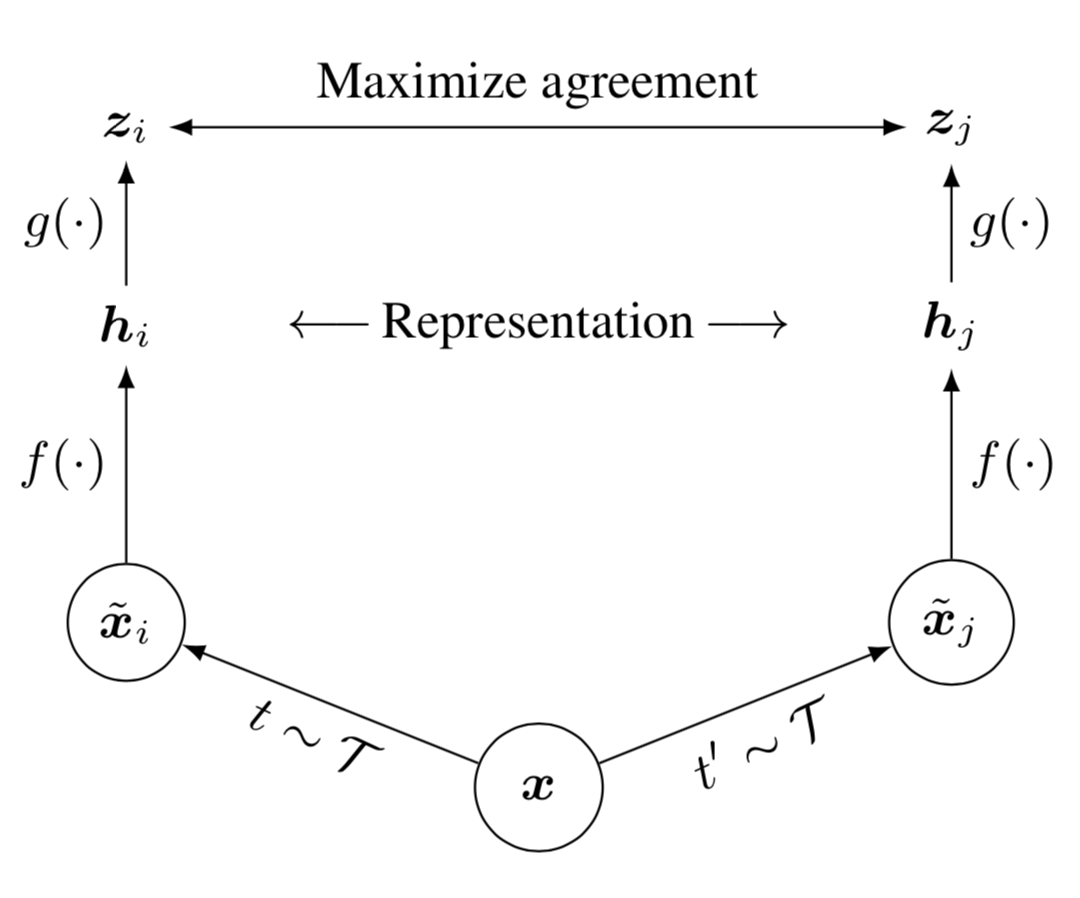Fig. 6. A simple framework for contrastive learning of visual representations. (Image source: Chen et al, 2020)

1) Randomly sample a minibatch of $$N$$ samples and each sample is applied with two different data augmentation operations, resulting in $$2N$$ augmented samples in total.

$\tilde{\mathbf{x}}_i = t(\mathbf{x}),\quad\tilde{\mathbf{x}}_j = t'(\mathbf{x}),\quad t, t' \sim \mathcal{T}$

where two separate data augmentation operators, $$t$$ and $$t'$$, are sampled from the same family of augmentations $$\mathcal{T}$$. Data augmentation includes random crop, resize with random flip, color distortions, and Gaussian blur.

2) Given one positive pair, other $$2(N-1)$$ data points are treated as negative samples. The representation is produced by a base encoder $$f(.)$$:

$\mathbf{h}_i = f(\tilde{\mathbf{x}}_i),\quad \mathbf{h}_j = f(\tilde{\mathbf{x}}_j)$

3) The contrastive learning loss is defined using cosine similarity $$\text{sim}(.,.)$$. Note that the loss operates on an extra projection layer of the representation $$g(.)$$ rather than on the representation space directly. But only the representation $$\mathbf{h}$$ is used for downstream tasks.

\begin{aligned} \mathbf{z}_i &= g(\mathbf{h}_i),\quad \mathbf{z}_j = g(\mathbf{h}_j) \\ \mathcal{L}_\text{SimCLR}^{(i,j)} &= - \log\frac{\exp(\text{sim}(\mathbf{z}_i, \mathbf{z}_j) / \tau)}{\sum_{k=1}^{2N} \mathbb{1}_{[k \neq i]} \exp(\text{sim}(\mathbf{z}_i, \mathbf{z}_k) / \tau)} \end{aligned}

where $$\mathbb{1}_{[k \neq i]}$$ is an indicator function: 1 if $$k\neq i$$ 0 otherwise.

SimCLR needs a large batch size to incorporate enough negative samples to achieve good performance.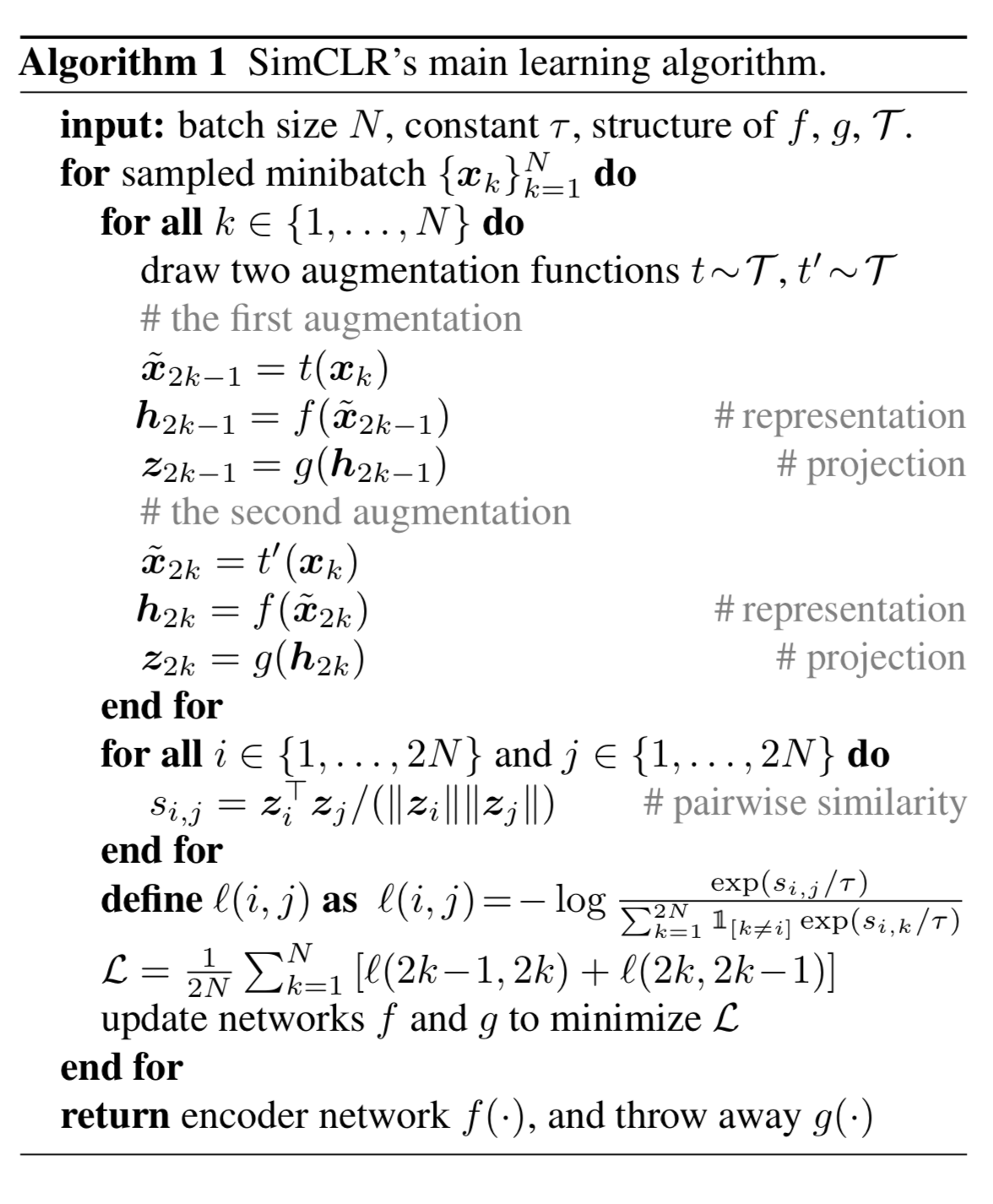Fig. 7. The algorithm for SimCLR. (Image source: Chen et al, 2020).

#### Barlow Twins

Barlow Twins (Zbontar et al. 2021) feeds two distorted versions of samples into the same network to extract features and learns to make the cross-correlation matrix between these two groups of output features close to the identity. The goal is to keep the representation vectors of different distorted versions of one sample similar, while minimizing the redundancy between these vectors.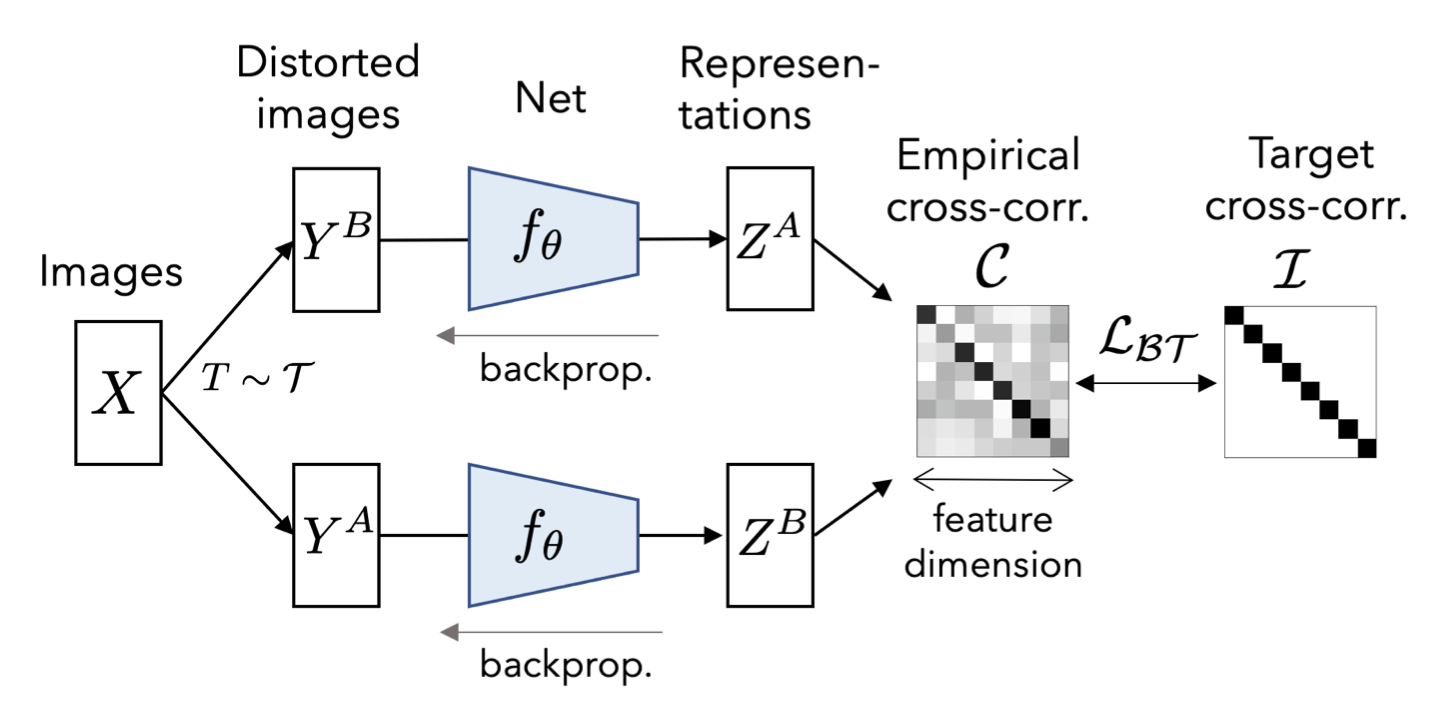Fig. 8. Illustration of Barlow Twins learning pipeline. (Image source: Zbontar et al. 2021).

Let $$\mathcal{C}$$ be a cross-correlation matrix computed between outputs from two identical networks along the batch dimension. $$\mathcal{C}$$ is a square matrix with the size same as the feature network’s output dimensionality. Each entry in the matrix $$\mathcal{C}_{ij}$$ is the cosine similarity between network output vector dimension at index $$i, j$$ and batch index $$b$$, $$\mathbf{z}_{b,i}^A$$ and $$\mathbf{z}_{b,j}^B$$, with a value between -1 (i.e. perfect anti-correlation) and 1 (i.e. perfect correlation).

\begin{aligned} \mathcal{L}_\text{BT} &= \underbrace{\sum_i (1-\mathcal{C}_{ii})^2}_\text{invariance term} + \lambda \underbrace{\sum_i\sum_{i\neq j} \mathcal{C}_{ij}^2}_\text{redundancy reduction term} \\ \text{where } \mathcal{C}_{ij} &= \frac{\sum_b \mathbf{z}^A_{b,i} \mathbf{z}^B_{b,j}}{\sqrt{\sum_b (\mathbf{z}^A_{b,i})^2}\sqrt{\sum_b (\mathbf{z}^B_{b,j})^2}} \end{aligned}

Barlow Twins is competitive with SOTA methods for self-supervised learning. It naturally avoids trivial constants (i.e. collapsed representations), and is robust to different training batch sizes.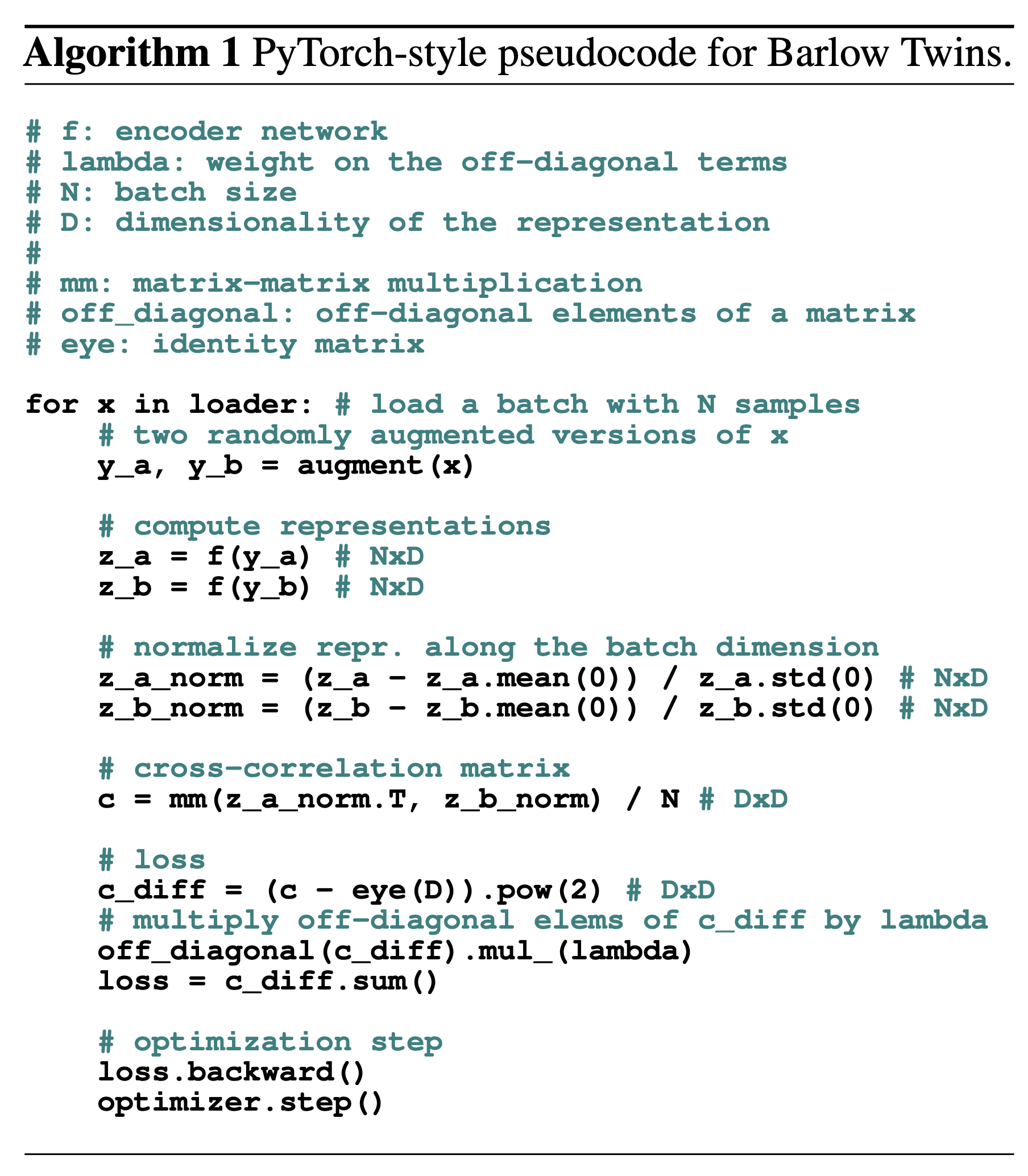Fig. 9. Algorithm of Barlow Twins in Pytorch style pseudo code. (Image source: Zbontar et al. 2021).

#### BYOL

Different from the above approaches, interestingly, BYOL (Bootstrap Your Own Latent; Grill, et al 2020) claims to achieve a new state-of-the-art results without using egative samples. It relies on two neural networks, referred to as online and target networks that interact and learn from each other. The target network (parameterized by $$\xi$$) has the same architecture as the online one (parameterized by $$\theta$$), but with polyak averaged weights, $$\xi \leftarrow \tau \xi + (1-\tau) \theta$$.

The goal is to learn a presentation $$y$$ that can be used in downstream tasks. The online network parameterized by $$\theta$$ contains:

• An encoder $$f_\theta$$;
• A projector $$g_\theta$$;
• A predictor $$q_\theta$$.

The target network has the same network architecture, but with different parameter $$\xi$$, updated by polyak averaging $$\theta$$: $$\xi \leftarrow \tau \xi + (1-\tau) \theta$$.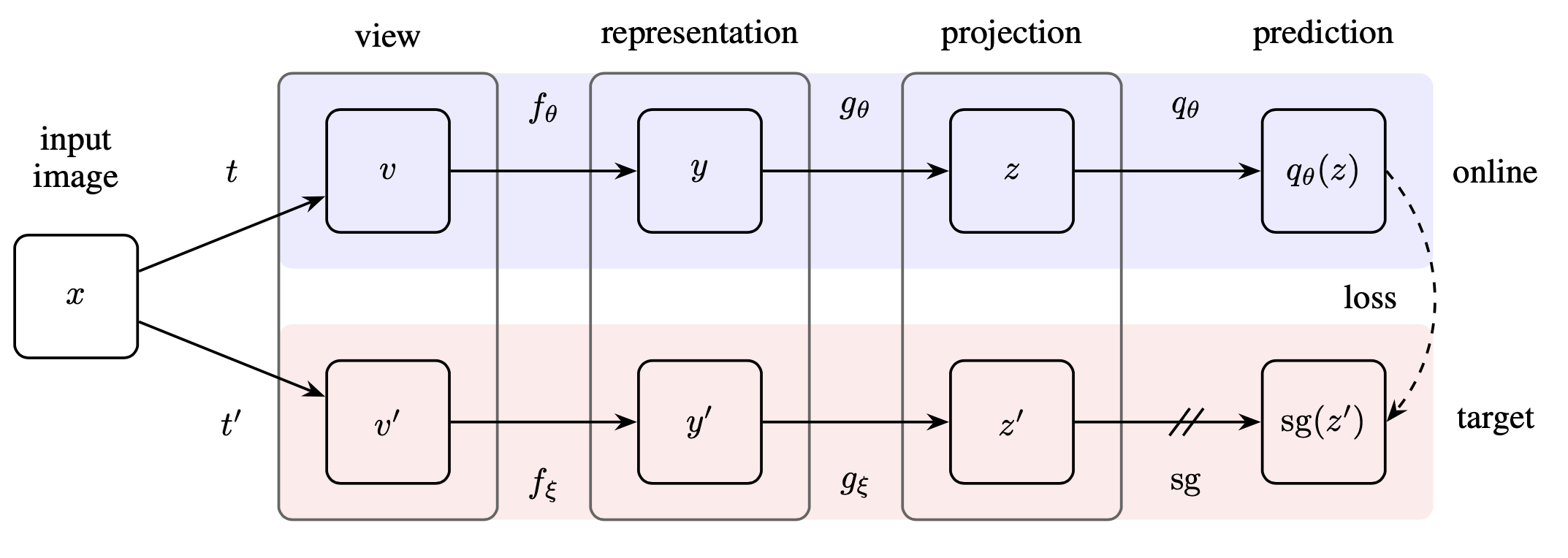Fig. 10. The model architecture of BYOL. After training, we only care about $$f_\theta$$ for producing representation, $$y=f_\theta(x)$$, and everything else is discarded. $$\text{sg}$$ means stop gradient. (Image source: Grill, et al 2020)

Given an image $$\mathbf{x}$$, the BYOL loss is constructed as follows:

• Create two augmented views: $$\mathbf{v}=t(\mathbf{x}); \mathbf{v}'=t'(\mathbf{x})$$ with augmentations sampled $$t \sim \mathcal{T}, t' \sim \mathcal{T}'$$;
• Then they are encoded into representations, $$\mathbf{y}_\theta=f_\theta(\mathbf{v}), \mathbf{y}'=f_\xi(\mathbf{v}')$$;
• Then they are projected into latent variables, $$\mathbf{z}_\theta=g_\theta(\mathbf{y}_\theta), \mathbf{z}'=g_\xi(\mathbf{y}')$$;
• The online network outputs a prediction $$q_\theta(\mathbf{z}_\theta)$$;
• Both $$q_\theta(\mathbf{z}_\theta)$$ and $$\mathbf{z}'$$ are L2-normalized, giving us $$\bar{q}_\theta(\mathbf{z}_\theta) = q_\theta(\mathbf{z}_\theta) / \| q_\theta(\mathbf{z}_\theta) \|$$ and $$\bar{\mathbf{z}'} = \mathbf{z}' / \|\mathbf{z}'\|$$;
• The loss $$\mathcal{L}^\text{BYOL}_\theta$$ is MSE between L2-normalized prediction $$\bar{q}_\theta(\mathbf{z})$$ and $$\bar{\mathbf{z}'}$$;
• The other symmetric loss $$\tilde{\mathcal{L}}^\text{BYOL}_\theta$$ can be generated by switching $$\mathbf{v}'$$ and $$\mathbf{v}$$; that is, feeding $$\mathbf{v}'$$ to online network and $$\mathbf{v}$$ to target network.
• The final loss is $$\mathcal{L}^\text{BYOL}_\theta + \tilde{\mathcal{L}}^\text{BYOL}_\theta$$ and only parameters $$\theta$$ are optimized.

Unlike most popular contrastive learning based approaches, BYOL does not use negative pairs. Most bootstrapping approaches rely on pseudo-labels or cluster indices, but BYOL directly boostrapps the latent representation.

It is quite interesting and surprising that without negative samples, BYOL still works well. Later I ran into this post by Abe Fetterman & Josh Albrecht, they highlighted two surprising findings while they were trying to reproduce BYOL:

1. BYOL generally performs no better than random when batch normalization is removed.
2. The presence of batch normalization implicitly causes a form of contrastive learning. They believe that using negative samples is important for avoiding model collapse (i.e. what if you use all-zeros representation for every data point?). Batch normalization injects dependency on negative samples inexplicitly because no matter how similar a batch of inputs are, the values are re-distributed (spread out $$\sim \mathcal{N}(0, 1$$) and therefore batch normalization prevents model collapse. Strongly recommend you to read the full article if you are working in this area.

### Memory Bank

Computing embeddings for a large number of negative samples in every batch is extremely expensive. One common approach is to store the representation in memory to trade off data staleness for cheaper compute.

#### Instance Discrimination with Memoy Bank

Instance contrastive learning (Wu et al, 2018) pushes the class-wise supervision to the extreme by considering each instance as a distinct class of its own. It implies that the number of “classes” will be the same as the number of samples in the training dataset. Hence, it is unfeasible to train a softmax layer with these many heads, but instead it can be approximated by NCE.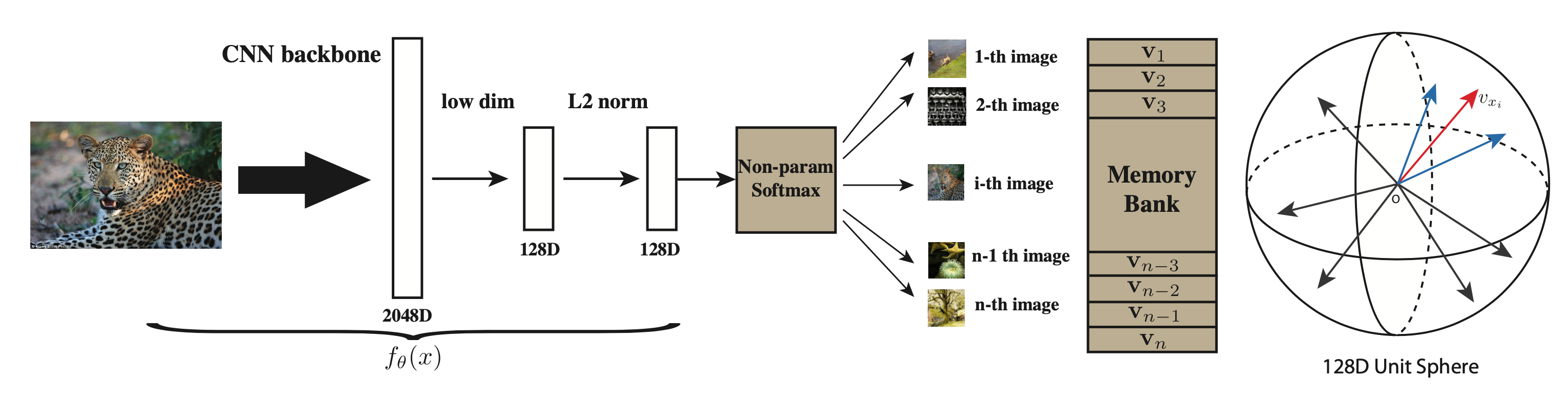Fig. 11. The training pipeline of instance-level contrastive learning. The learned embedding is L2-normalized. (Image source: Wu et al, 2018)

Let $$\mathbf{v} = f_\theta(x)$$ be an embedding function to learn and the vector is normalized to have $$\|\mathbf{v}\|=1$$. A non-parametric classifier predicts the probability of a sample $$\mathbf{v}$$ belonging to class $$i$$ with a temperature parameter $$\tau$$:

$P(C=i\vert \mathbf{v}) = \frac{\exp(\mathbf{v}_i^\top \mathbf{v} / \tau)}{\sum_{j=1}^n \exp(\mathbf{v}_j^\top \mathbf{v} / \tau)}$

Instead of computing the representations for all the samples every time, they implement an Memory Bank for storing sample representation in the database from past iterations. Let $$V=\{ \mathbf{v}_i \}$$ be the memory bank and $$\mathbf{f}_i = f_\theta(\mathbf{x}_i)$$ be the feature generated by forwarding the network. We can use the representation from the memory bank $$\mathbf{v}_i$$ instead of the feature forwarded from the network $$\mathbf{f}_i$$ when comparing pairwise similarity.

The denominator theoretically requires access to the representations of all the samples, but that is too expensive in practice. Instead we can estimate it via Monte Carlo approximation using a random subset of $$M$$ indices $$\{j_k\}_{k=1}^M$$.

$P(i\vert \mathbf{v}) = \frac{\exp(\mathbf{v}^\top \mathbf{f}_i / \tau)}{\sum_{j=1}^N \exp(\mathbf{v}_j^\top \mathbf{f}_i / \tau)} \simeq \frac{\exp(\mathbf{v}^\top \mathbf{f}_i / \tau)}{\frac{N}{M} \sum_{k=1}^M \exp(\mathbf{v}_{j_k}^\top \mathbf{f}_i / \tau)}$

Because there is only one instance per class, the training is unstable and fluctuates a lot. To improve the training smoothness, they introduced an extra term for positive samples in the loss function based on the proximal optimization method. The final NCE loss objective looks like:

\begin{aligned} \mathcal{L}_\text{instance} &= - \mathbb{E}_{P_d}\big[\log h(i, \mathbf{v}^{(t-1)}_i) - \lambda \|\mathbf{v}^{(t)}_i - \mathbf{v}^{(t-1)}_i\|^2_2\big] - M\mathbb{E}_{P_n}\big[\log(1 - h(i, \mathbf{v}'^{(t-1)})\big] \\ h(i, \mathbf{v}) &= \frac{P(i\vert\mathbf{v})}{P(i\vert\mathbf{v}) + MP_n(i)} \text{ where the noise distribution is uniform }P_n = 1/N \end{aligned}

where $$\{ \mathbf{v}^{(t-1)} \}$$ are embeddings stored in the memory bank from the previous iteration. The difference between iterations $$\|\mathbf{v}^{(t)}_i - \mathbf{v}^{(t-1)}_i\|^2_2$$ will gradually vanish as the learned embedding converges.

#### MoCo & MoCo-V2

Momentum Contrast (MoCo; He et al, 2019) provides a framework of unsupervised learning visual representation as a dynamic dictionary look-up. The dictionary is structured as a large FIFO queue of encoded representations of data samples.

Given a query sample $$\mathbf{x}_q$$, we get a query representation through an encoder $$\mathbf{q} = f_q(\mathbf{x}_q)$$. A list of key representations $$\{\mathbf{k}_1, \mathbf{k}_2, \dots \}$$ in the dictionary are encoded by a momentum encoder $$\mathbf{k}_i = f_k (\mathbf{x}^k_i)$$. Let’s assume among them there is a single positive key $$\mathbf{k}^+$$ in the dictionary that matches $$\mathbf{q}$$. In the paper, they create $$\mathbf{k}^+$$ using a noise copy of $$\mathbf{x}_q$$ with different augmentation. Then the InfoNCE contrastive loss with temperature $$\tau$$ is used over one positive and $$N-1$$ negative samples:

$\mathcal{L}_\text{MoCo} = - \log \frac{\exp(\mathbf{q} \cdot \mathbf{k}^+ / \tau)}{\sum_{i=1}^N \exp(\mathbf{q} \cdot \mathbf{k}_i / \tau)}$

Compared to the memory bank, a queue-based dictionary in MoCo enables us to reuse representations of immediately preceding mini-batches of data.

The MoCo dictionary is not differentiable as a queue, so we cannot rely on back-propagation to update the key encoder $$f_k$$. One naive way might be to use the same encoder for both $$f_q$$ and $$f_k$$. Differently, MoCo proposed to use a momentum-based update with a momentum coefficient $$m \in [0, 1)$$. Say, the parameters of $$f_q$$ and $$f_k$$ are labeled as $$\theta_q$$ and $$\theta_k$$, respectively.

$\theta_k \leftarrow m \theta_k + (1-m) \theta_q$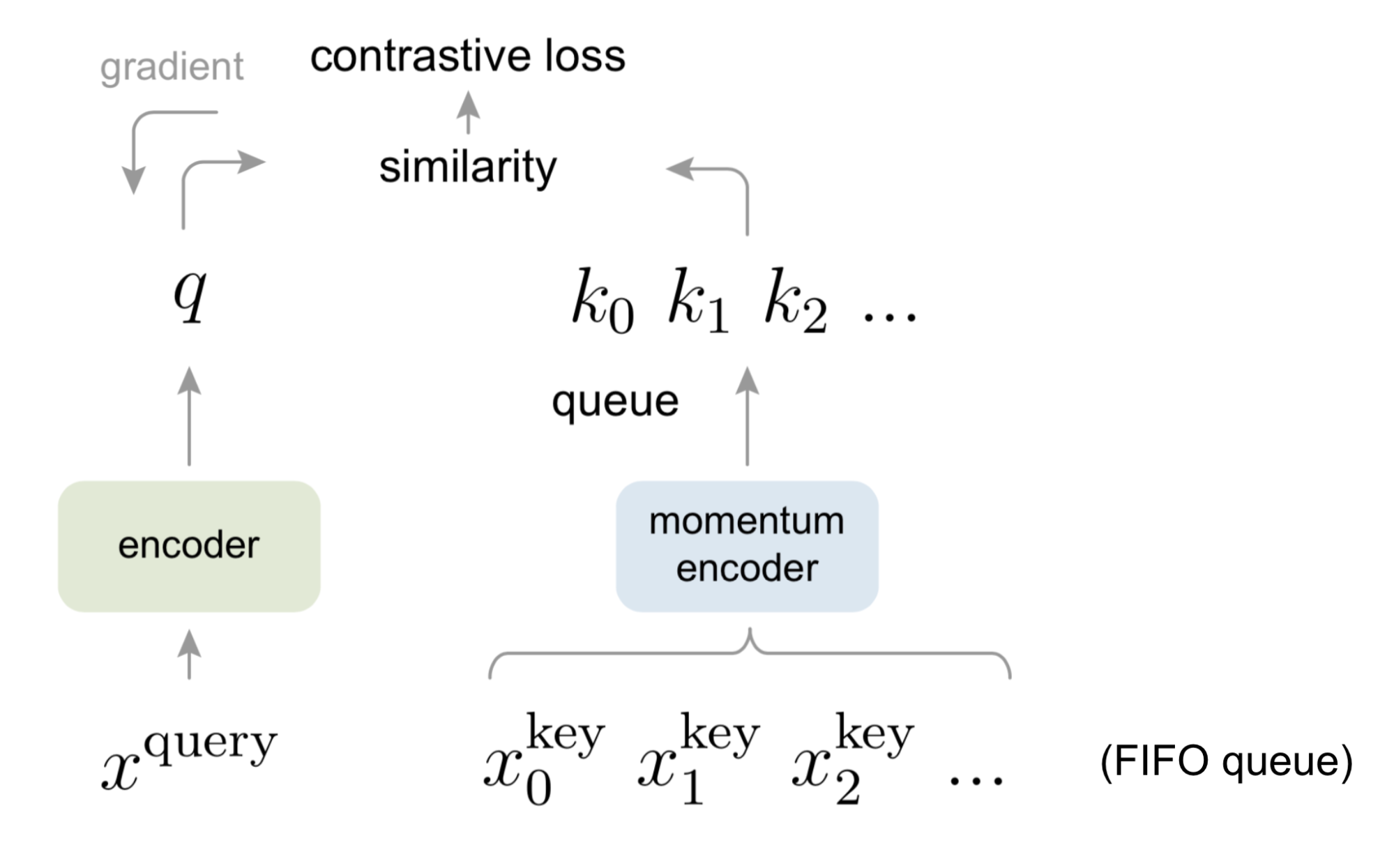Fig. 12. Illustration of how Momentum Contrast (MoCo) learns visual representations. (Image source: He et al, 2019)

The advantage of MoCo compared to SimCLR is that MoCo decouples the batch size from the number of negatives, but SimCLR requires a large batch size in order to have enough negative samples and suffers performance drops when their batch size is reduced.

Two designs in SimCLR, namely, (1) an MLP projection head and (2) stronger data augmentation, are proved to be very efficient. MoCo V2 (Chen et al, 2020) combined these two designs, achieving even better transfer performance with no dependency on a very large batch size.

#### CURL

CURL (Srinivas, et al. 2020) applies the above ideas in Reinforcement Learning. It learns a visual representation for RL tasks by matching embeddings of two data-augmented versions, $$o_q$$ and $$o_k$$, of the raw observation $$o$$ via contrastive loss. CURL primarily relies on random crop data augmentation. The key encoder is implemented as a momentum encoder with weights as EMA of the query encoder weights, same as in MoCo.

One significant difference between RL and supervised visual tasks is that RL depends on temporal consistency between consecutive frames. Therefore, CURL applies augmentation consistently on each stack of frames to retain information about the temporal structure of the observation.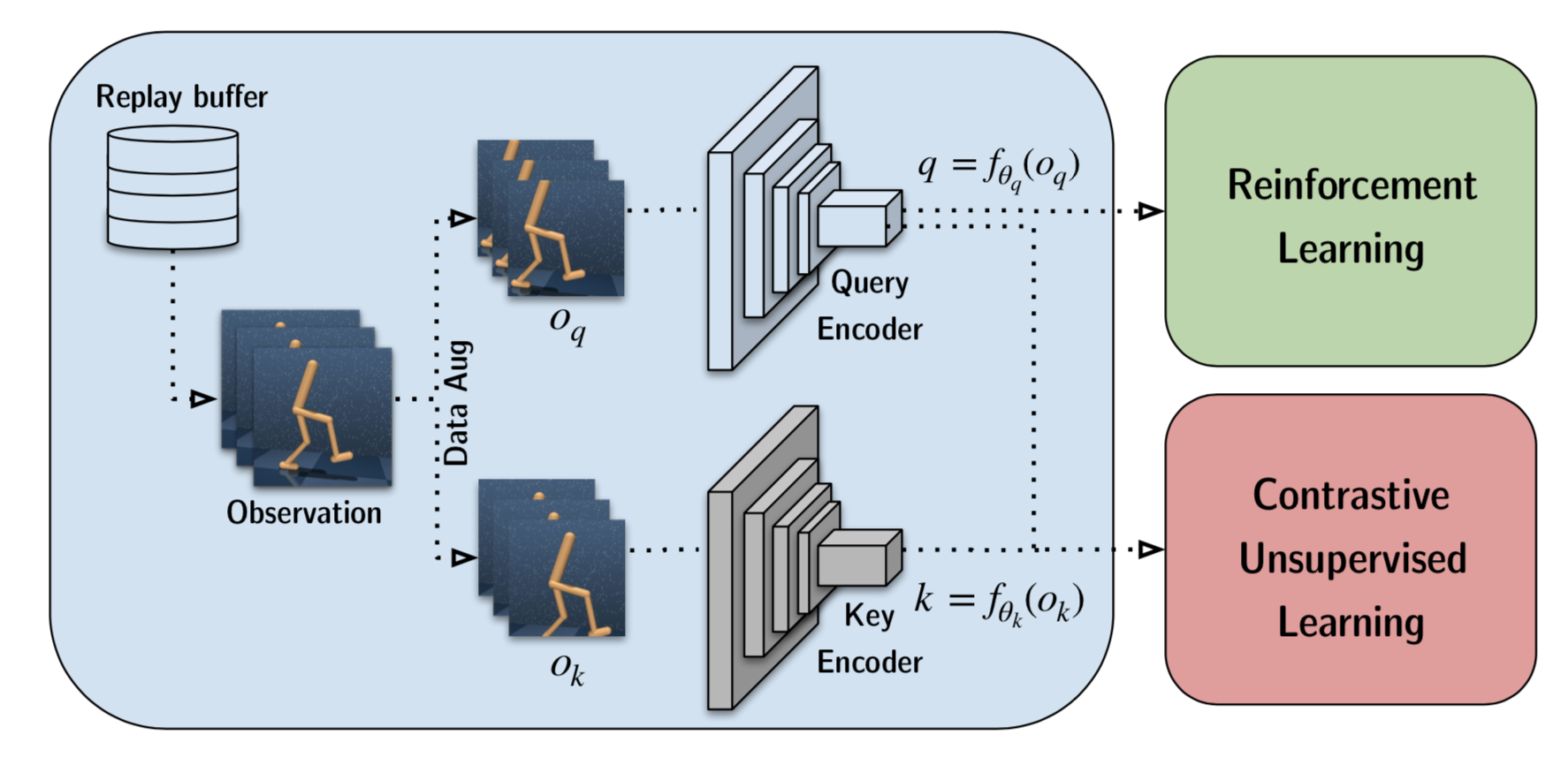Fig. 13. The architecture of CURL. (Image source: Srinivas, et al. 2020)

### Feature Clustering

#### DeepCluster

DeepCluster (Caron et al. 2018) iteratively clusters features via k-means and uses cluster assignments as pseudo labels to provide supervised signals.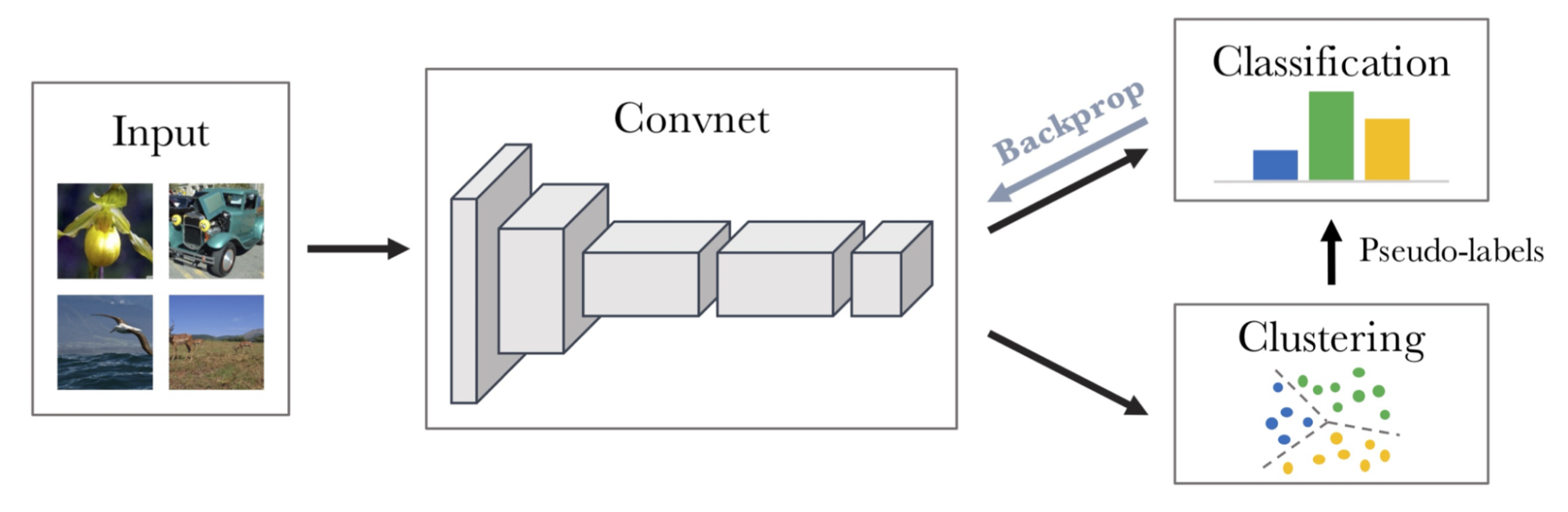Fig. 14. Illustration of DeepCluster method which iteratively clusters deep features and uses the cluster assignments as pseudo-labels. (Image source: Caron et al. 2018)

In each iteration, DeepCluster clusters data points using the prior representation and then produces the new cluster assignments as the classification targets for the new representation. However this iterative process is prone to trivial solutions. While avoiding the use of negative pairs, it requires a costly clustering phase and specific precautions to avoid collapsing to trivial solutions.

#### SwAV

SwAV (Swapping Assignments between multiple Views; Caron et al. 2020) is an online contrastive learning algorithm. It computes a code from an augmented version of the image and tries to predict this code using another augmented version of the same image.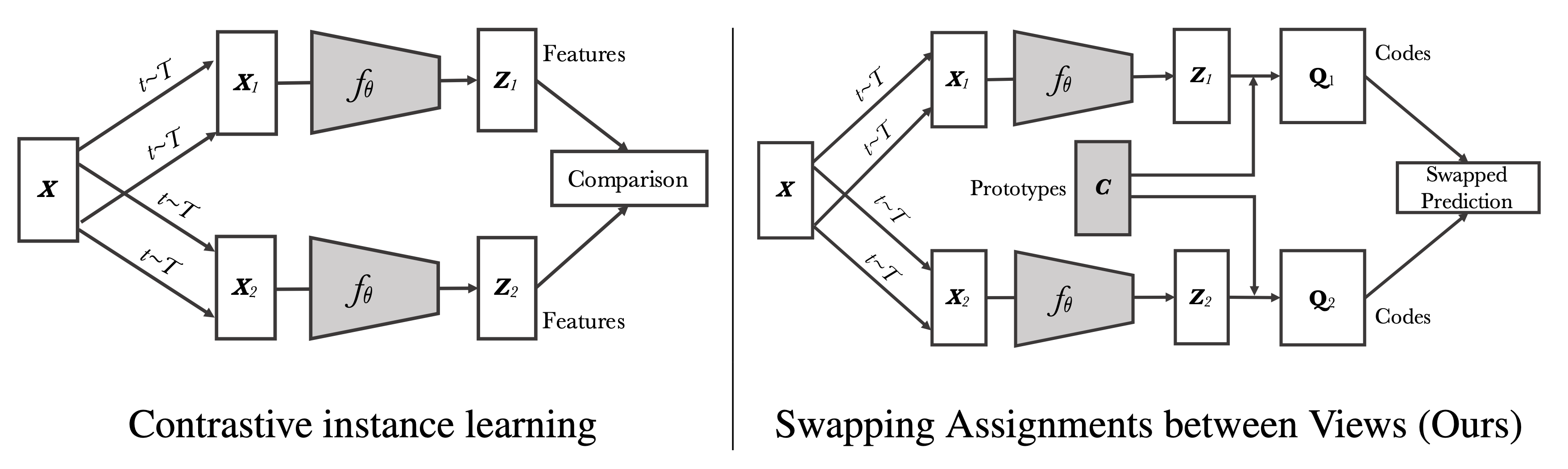Fig. 15. Comparison of SwAV and contrastive instance learning. (Image source: Caron et al. 2020)

Given features of images with two different augmentations, $$\mathbf{z}_t$$ and $$\mathbf{z}_s$$, SwAV computes corresponding codes $$\mathbf{q}_t$$ and $$\mathbf{q}_s$$ and the loss quantifies the fit by swapping two codes using $$\ell(.)$$ to measure the fit between a feature and a code.

$\mathcal{L}_\text{SwAV}(\mathbf{z}_t, \mathbf{z}_s) = \ell(\mathbf{z}_t, \mathbf{q}_s) + \ell(\mathbf{z}_s, \mathbf{q}_t)$

The swapped fit prediction depends on the cross entropy between the predicted code and a set of $$K$$ trainable prototype vectors $$\mathbf{C} = \{\mathbf{c}_1, \dots, \mathbf{c}_K\}$$. The prototype vector matrix is shared across different batches and represents anchor clusters that each instance should be clustered to.

$\ell(\mathbf{z}_t, \mathbf{q}_s) = - \sum_k \mathbf{q}^{(k)}_s\log\mathbf{p}^{(k)}_t \text{ where } \mathbf{p}^{(k)}_t = \frac{\exp(\mathbf{z}_t^\top\mathbf{c}_k / \tau)}{\sum_{k'}\exp(\mathbf{z}_t^\top \mathbf{c}_{k'} / \tau)}$

In a mini-batch containing $$B$$ feature vectors $$\mathbf{Z} = [\mathbf{z}_1, \dots, \mathbf{z}_B]$$, the mapping matrix between features and prototype vectors is defined as $$\mathbf{Q} = [\mathbf{q}_1, \dots, \mathbf{q}_B] \in \mathbb{R}_+^{K\times B}$$. We would like to maximize the similarity between the features and the prototypes:

\begin{aligned} \max_{\mathbf{Q}\in\mathcal{Q}} &\text{Tr}(\mathbf{Q}^\top \mathbf{C}^\top \mathbf{Z}) + \varepsilon \mathcal{H}(\mathbf{Q}) \\ \text{where }\mathcal{Q} &= \big\{ \mathbf{Q} \in \mathbb{R}_{+}^{K \times B} \mid \mathbf{Q}\mathbf{1}_B = \frac{1}{K}\mathbf{1}_K, \mathbf{Q}^\top\mathbf{1}_K = \frac{1}{B}\mathbf{1}_B \big\} \end{aligned}

where $$\mathcal{H}$$ is the entropy, $$\mathcal{H}(\mathbf{Q}) = - \sum_{ij} \mathbf{Q}_{ij} \log \mathbf{Q}_{ij}$$, controlling the smoothness of the code. The coefficient $$\epsilon$$ should not be too large; otherwise, all the samples will be assigned uniformly to all the clusters. The candidate set of solutions for $$\mathbf{Q}$$ requires every mapping matrix to have each row sum up to $$1/K$$ and each column to sum up to $$1/B$$, enforcing that each prototype gets selected at least $$B/K$$ times on average.

SwAV relies on the iterative Sinkhorn-Knopp algorithm (Cuturi 2013) to find the solution for $$\mathbf{Q}$$.

### Working with Supervised Datasets

#### CLIP

CLIP (Contrastive Language-Image Pre-training; Radford et al. 2021) jointly trains a text encoder and an image feature extractor over the pretraining task that predicts which caption goes with which image.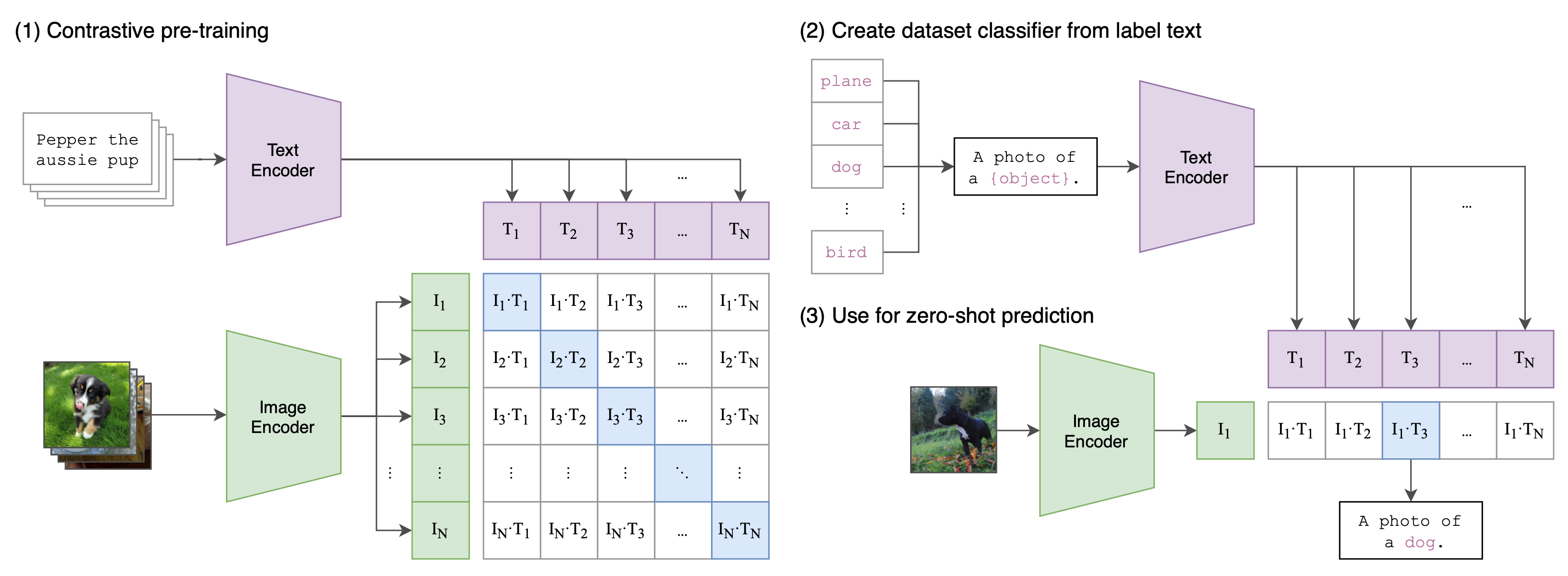Fig. 16. Illustration of CLIP contrastive pre-training over text-image pairs. (Image source: Radford et al. 2021)

Given a batch of $$N$$ (image, text) pairs, CLIP computes the dense cosine similarity matrix between all $$N\times N$$ possible (image, text) candidates within this batch. The text and image encoders are jointly trained to maximize the similarity between $$N$$ correct pairs of (image, text) associations while minimizing the similarity for $$N(N-1)$$ incorrect pairs via a symmetric cross entropy loss over the dense matrix.

See the numy-like pseudo code for CLIP in Fig. 17.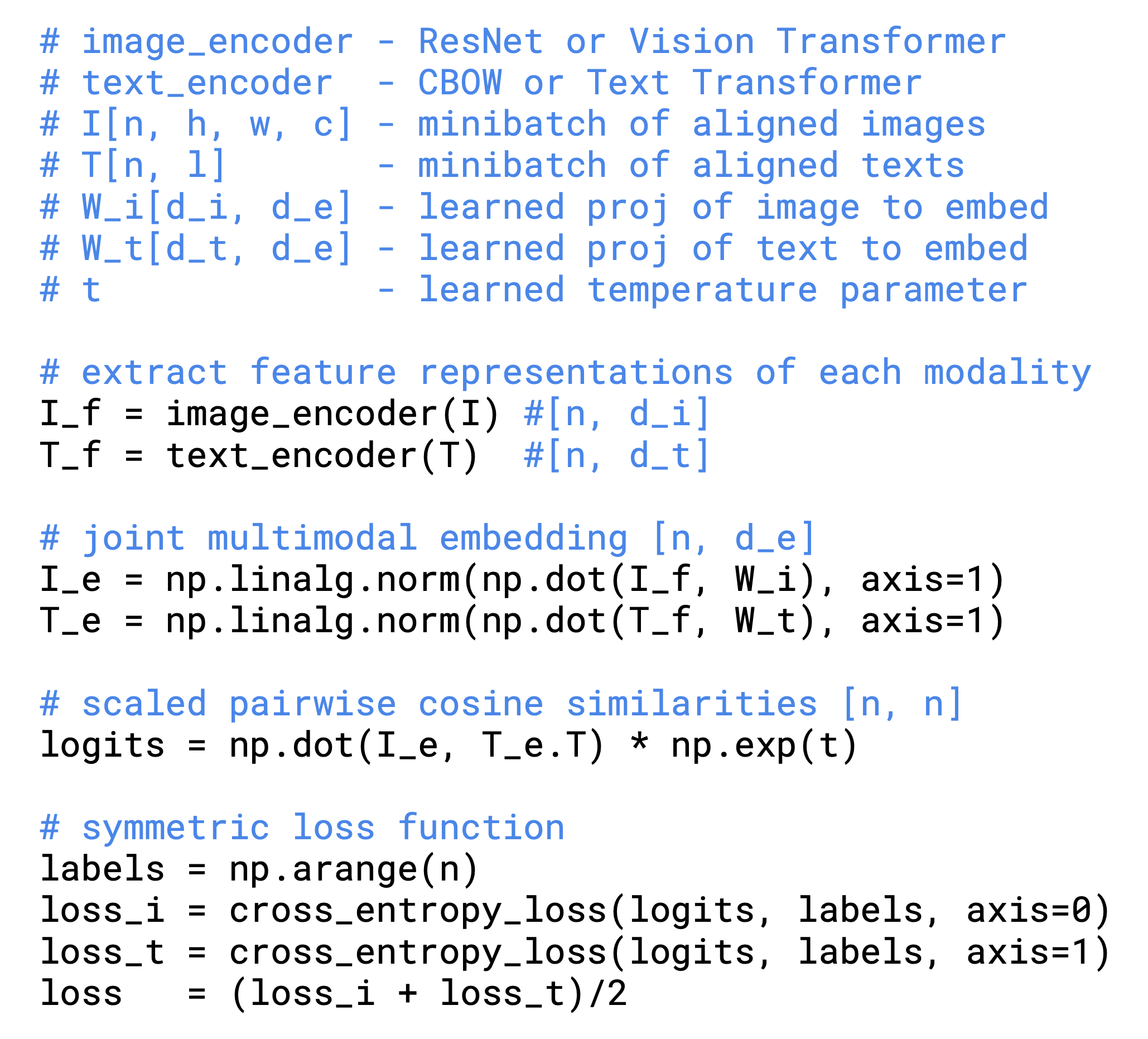Fig. 17. CLIP algorithm in Numpy style pseudo code. (Image source: Radford et al. 2021)

Compared to other methods above for learning good visual representation, what makes CLIP really special is “the appreciation of using natural language as a training signal”. It does demand access to supervised dataset in which we know which text matches which image. It is trained on 400 million (text, image) pairs, collected from the Internet. The query list contains all the words occurring at least 100 times in the English version of Wikipedia. Interestingly, they found that Transformer-based language models are 3x slower than a bag-of-words (BoW) text encoder at zero-shot ImageNet classification. Using contrastive objective instead of trying to predict the exact words associated with images (i.e. a method commonly adopted by image caption prediction tasks) can further improve the data efficiency another 4x.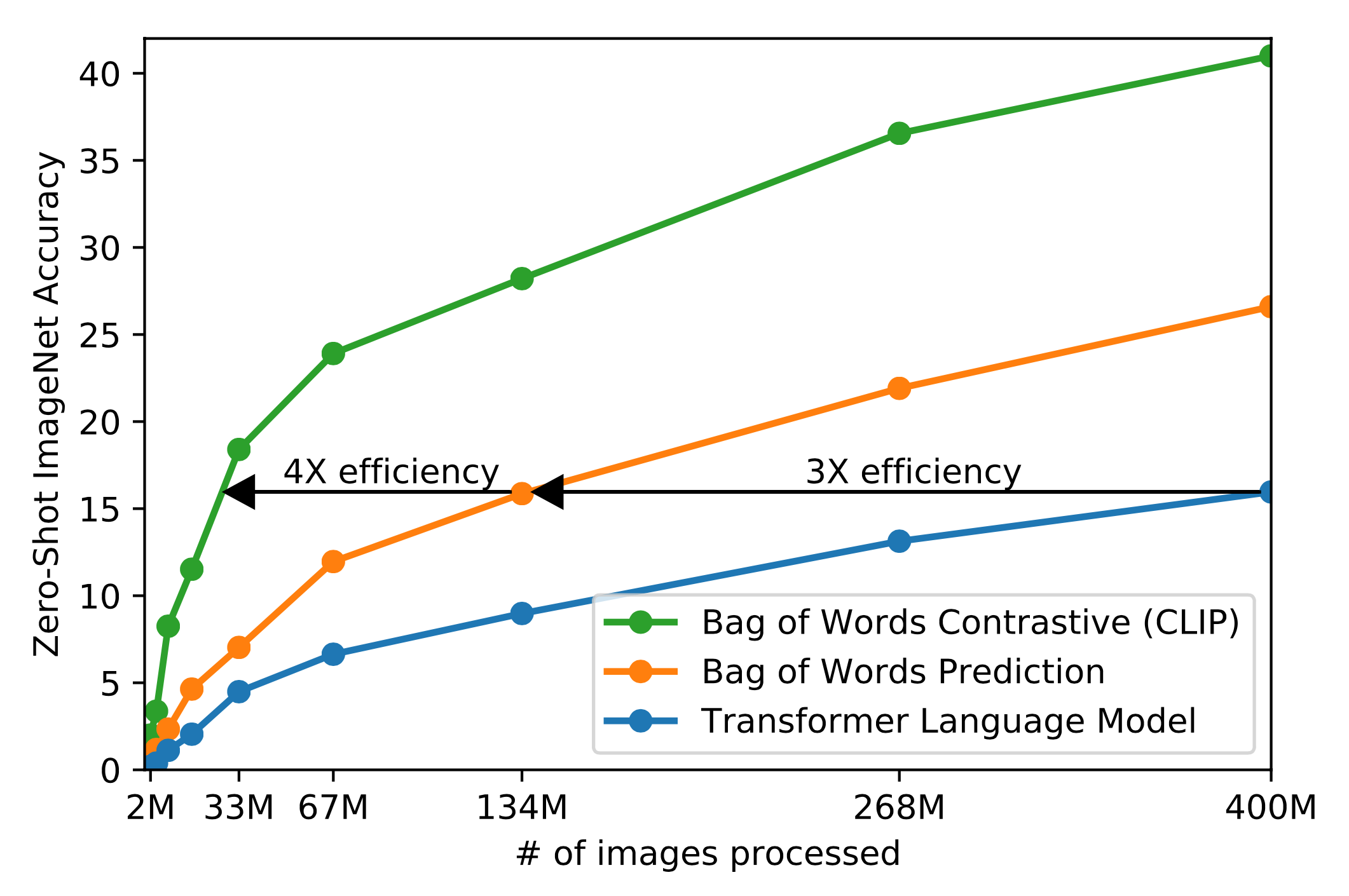Fig. 18. Using bag-of-words text encoding and contrastive training objectives can bring in multiple folds of data efficiency improvement. (Image source: Radford et al. 2021)

CLIP produces good visual representation that can non-trivially transfer to many CV benchmark datasets, achieving results competitive with supervised baseline. Among tested transfer tasks, CLIP struggles with very fine-grained classification, as well as abstract or systematic tasks such as counting the number of objects. The transfer performance of CLIP models is smoothly correlated with the amount of model compute.

#### Supervised Contrastive Learning

There are several known issues with cross entropy loss, such as the lack of robustness to noisy labels and the possibility of poor margins. Existing improvement for cross entropy loss involves the curation of better training data, such as label smoothing and data augmentation. Supervised Contrastive Loss (Khosla et al. 2021) aims to leverage label information more effectively than cross entropy, imposing that normalized embeddings from the same class are closer together than embeddings from different classes.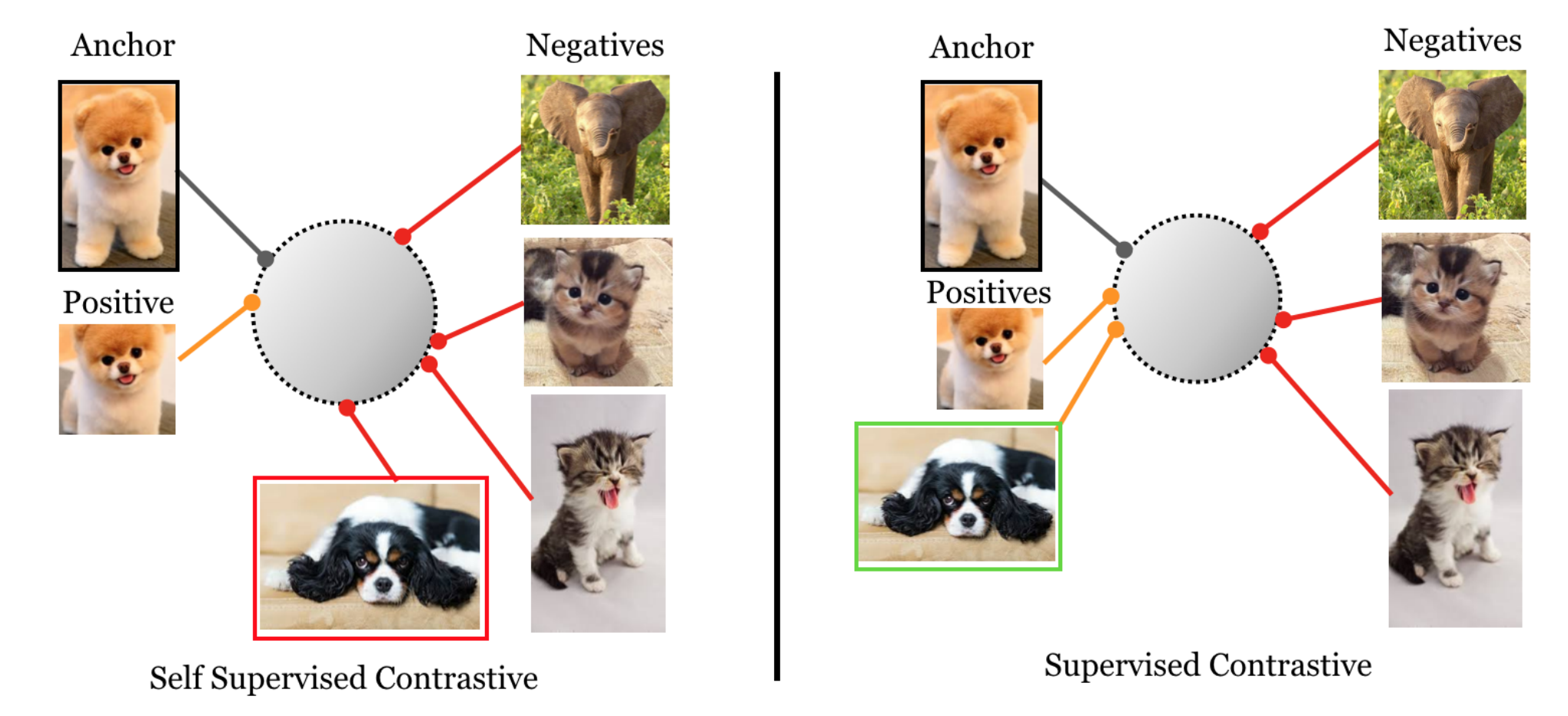Fig. 19. Supervised vs self-supervised contrastive losses. Supervised contrastive learning considers different samples from the same class as positive examples, in addition to augmented versions. (Image source: Khosla et al. 2021)

Given a set of randomly sampled $$n$$ (image, label) pairs, $$\{\mathbf{x}_i, y_i\}_{i=1}^n$$, $$2n$$ training pairs can be created by applying two random augmentations of every sample, $$\{\tilde{\mathbf{x}}_i, \tilde{y}_i\}_{i=1}^{2n}$$.

Supervised contrastive loss $$\mathcal{L}_\text{supcon}$$ utilizes multiple positive and negative samples, very similar to soft nearest-neighbor loss:

$\mathcal{L}_\text{supcon} = - \sum_{i=1}^{2n} \frac{1}{2 \vert N_i \vert - 1} \sum_{j \in N(y_i), j \neq i} \log \frac{\exp(\mathbf{z}_i \cdot \mathbf{z}_j / \tau)}{\sum_{k \in I, k \neq i}\exp({\mathbf{z}_i \cdot \mathbf{z}_k / \tau})}$

where $$\mathbf{z}_k=P(E(\tilde{\mathbf{x}_k}))$$, in which $$E(.)$$ is an encoder network (augmented image mapped to vector) $$P(.)$$ is a projection network (one vector mapped to another). $$N_i= \{j \in I: \tilde{y}_j = \tilde{y}_i \}$$ contains a set of indices of samples with label $$y_i$$. Including more positive samples into the set $N_i$ leads to improved results.

According to their experiments, supervised contrastive loss:

• does outperform the base cross entropy, but only by a small amount.
• outperforms the cross entropy on robustness benchmark (ImageNet-C, which applies common naturally occuring perturbations such as noise, blur and contrast changes to the ImageNet dataset).
• is less sensitive to hyperparameter changes.

## Language: Sentence Embedding

In this section, we focus on how to learn sentence embedding.

### Text Augmentation

Most contrastive methods in vision applications depend on creating an augmented version of each image. However, it is more challenging to construct text augmentation which does not alter the semantics of a sentence. In this section we look into three approaches for augmenting text sequences, including lexical edits, back-translation and applying cutoff or dropout.

#### Lexical Edits

EDA (Easy Data Augmentation; Wei & Zou 2019) defines a set of simple but powerful operations for text augmentation. Given a sentence, EDA randomly chooses and applies one of four simple operations:

1. Synonym replacement (SR): Replace $$n$$ random non-stop words with their synonyms.
2. Random insertion (RI): Place a random synonym of a randomly selected non-stop word in the sentence at a random position.
3. Random swap (RS): Randomly swap two words and repeat $$n$$ times.
4. Random deletion (RD): Randomly delete each word in the sentence with probability $$p$$.

where $$p=\alpha$$ and $$n=\alpha \times \text{sentence_length}$$, with the intuition that longer sentences can absorb more noise while maintaining the original label. The hyperparameter $$\alpha$$ roughly indicates the percent of words in one sentence that may be changed by one augmentation.

EDA is shown to improve the classification accuracy on several classification benchmark datasets compared to baseline without EDA. The performance lift is more significant on a smaller training set. All the four operations in EDA help improve the classification accuracy, but get to optimal at different $$\alpha$$’s.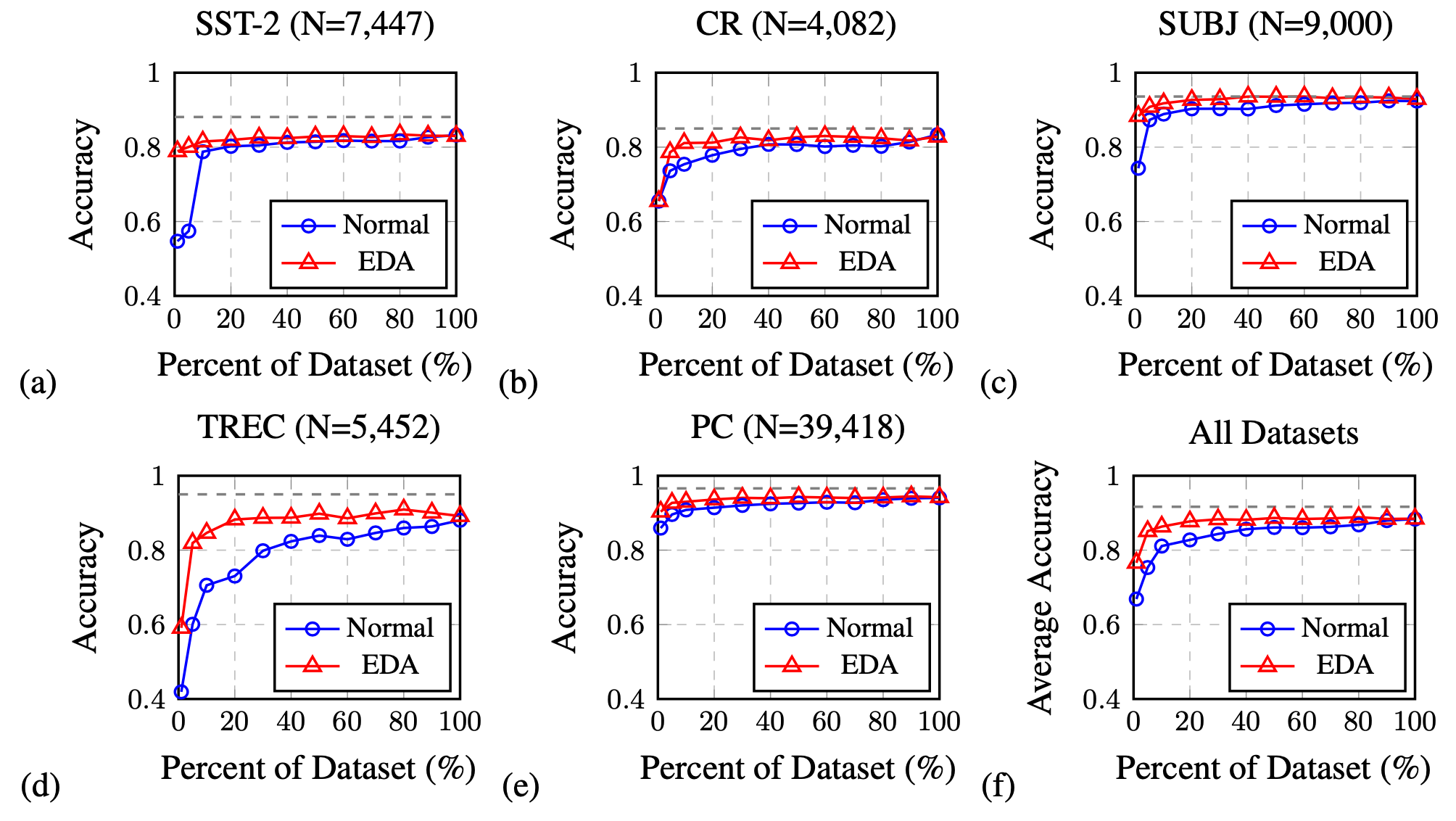Fig. 20. EDA leads to performance improvement on several classification benchmarks. (Image source: Wei & Zou 2019)

In Contextual Augmentation (Sosuke Kobayashi, 2018), new substitutes for word $$w_i$$ at position $$i$$ can be smoothly sampled from a given probability distribution, $$p(.\mid S\setminus\{w_i\})$$, which is predicted by a bidirectional LM like BERT.

#### Back-translation

CERT (Contrastive self-supervised Encoder Representations from Transformers; Fang et al. (2020); code) generates augmented sentences via back-translation. Various translation models for different languages can be employed for creating different versions of augmentations. Once we have a noise version of text samples, many contrastive learning frameworks introduced above, such as MoCo, can be used to learn sentence embedding.

#### Dropout and Cutoff

Shen et al. (2020) proposed to apply Cutoff to text augmentation, inspired by cross-view training. They proposed three cutoff augmentation strategies:

1. Token cutoff removes the information of a few selected tokens. To make sure there is no data leakage, corresponding tokens in the input, positional and other relevant embedding matrices should all be zeroed out.,
2. Feature cutoff removes a few feature columns.
3. Span cutoff removes a continuous chunk of texts.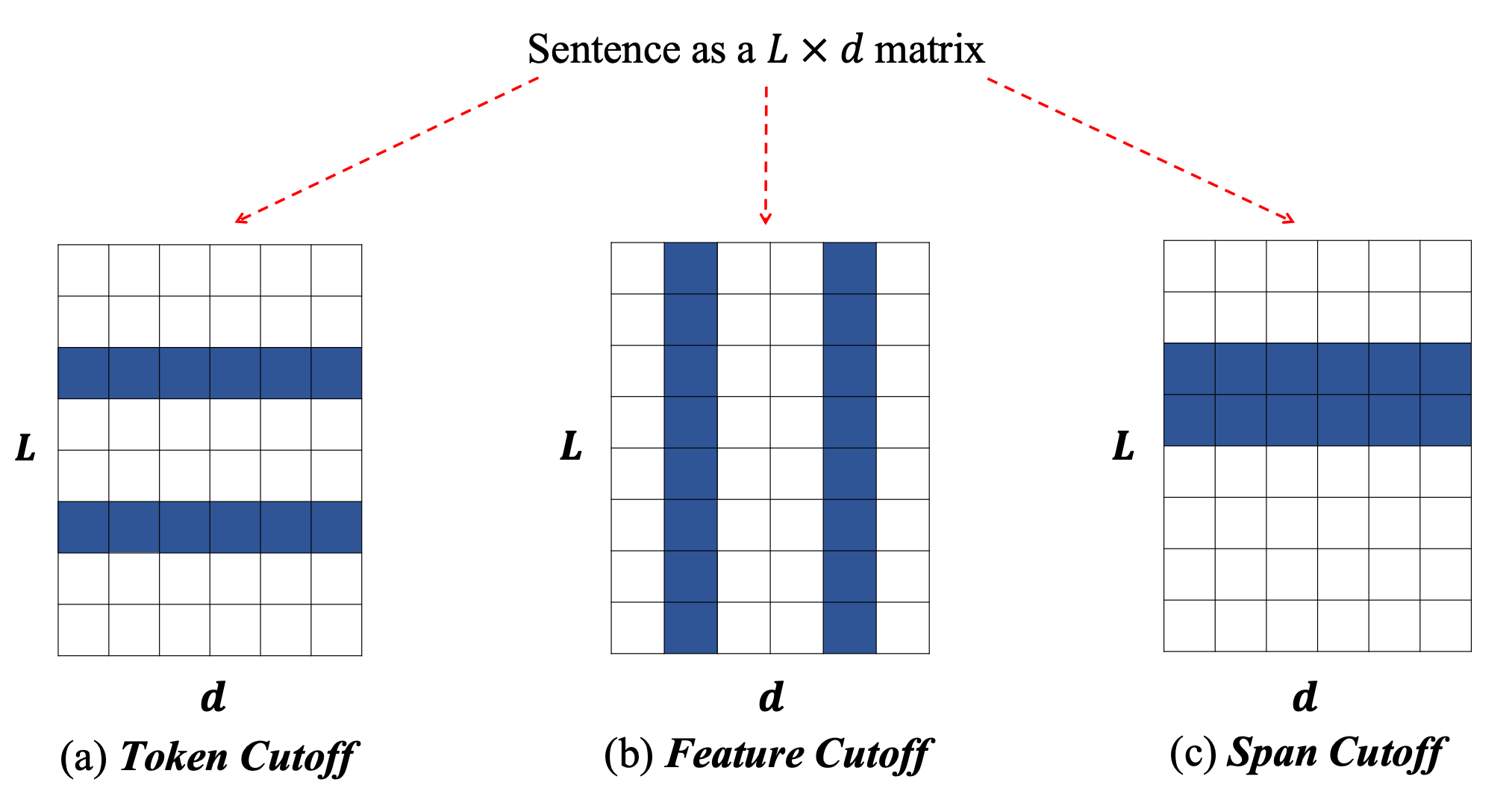Fig. 21. Schematic illustration of token, feature and span cutoff augmentation strategies. (Image source: Shen et al. 2020)

Multiple augmented versions of one sample can be created. When training, Shen et al. (2020) applied an additional KL-divergence term to measure the consensus between predictions from different augmented samples.

SimCSE (Gao et al. 2021; code) learns from unsupervised data by predicting a sentence from itself with only dropout noise. In other words, they treat dropout as data augmentation for text sequences. A sample is simply fed into the encoder twice with different dropout masks and these two versions are the positive pair where the other in-batch samples are considered as negative pairs. It feels quite similar to the cutoff augmentation, but dropout is more flexible with less well-defined semantic meaning of what content can be masked off.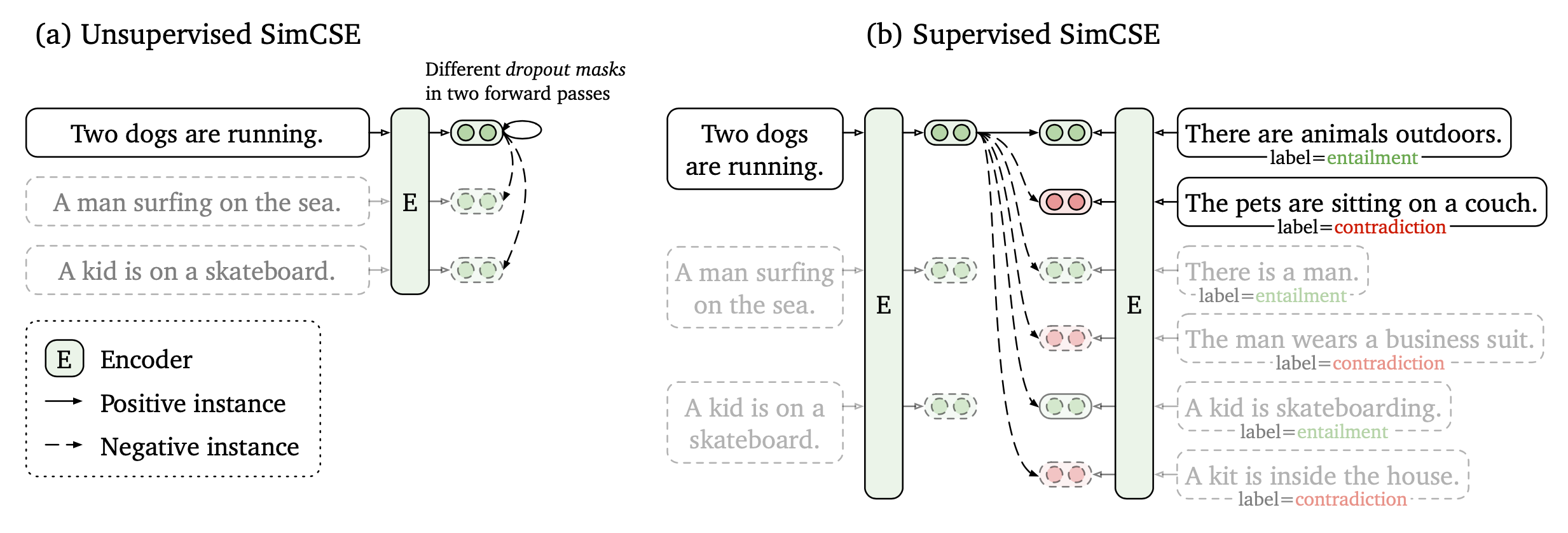Fig. 22. SimCSE creates augmented samples by applying different dropout masks. The supervised version leverages NLI datasets to predict positive (entailment) or negative (contradiction) given a pair of sentences. (Image source: Gao et al. 2021)

They ran experiments on 7 STS (Semantic Text Similarity) datasets and computed cosine similarity between sentence embeddings. They also tried out an optional MLM auxiliary objective loss to help avoid catastrophic forgetting of token-level knowledge. This aux loss was found to help improve performance on transfer tasks, but a consistent drop on the main STS tasks.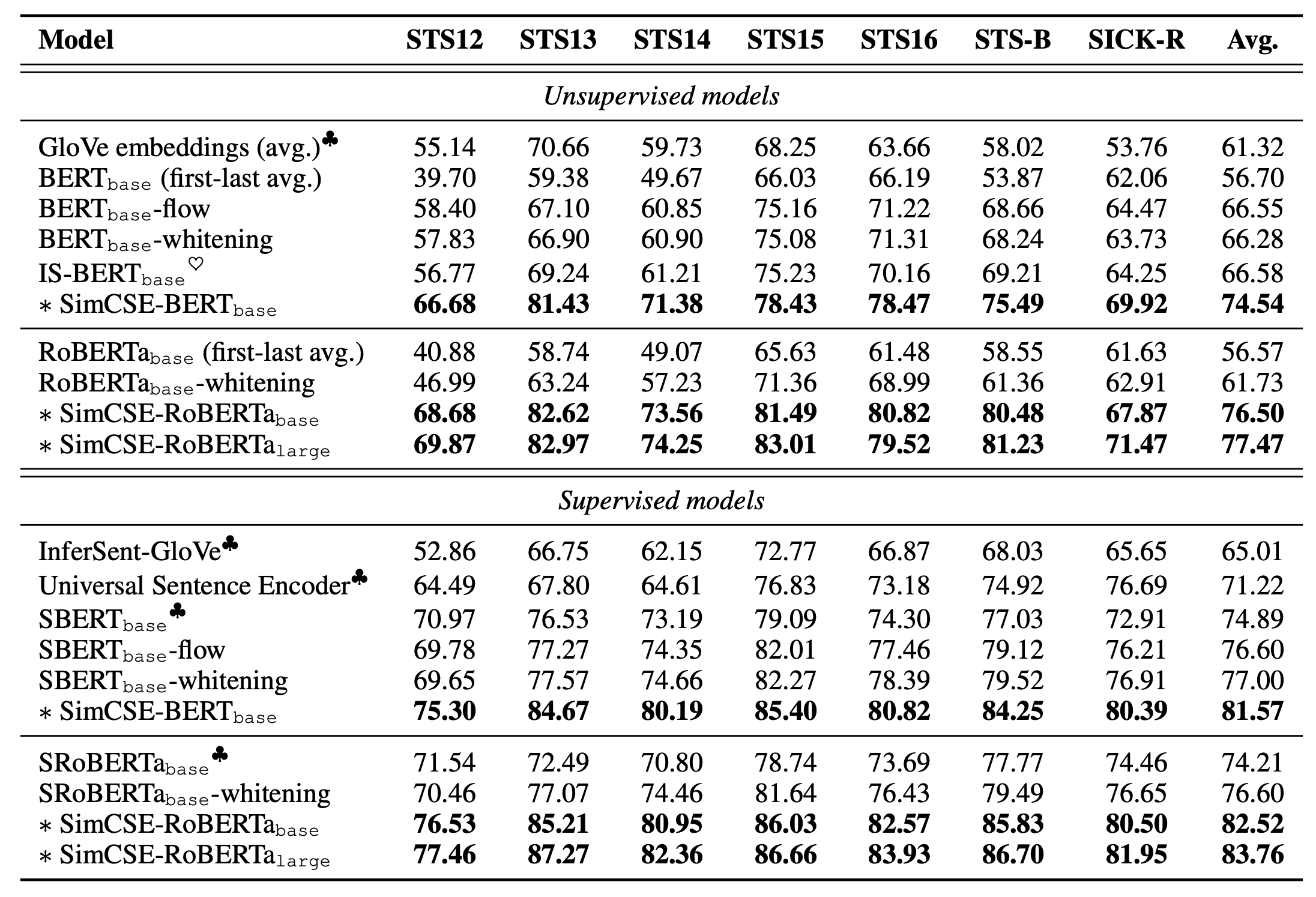Fig. 23. Experiment numbers on a collection of STS benchmarks with SimCES. (Image source: Gao et al. 2021)

### Supervision from NLI

The pre-trained BERT sentence embedding without any fine-tuning has been found to have poor performance for semantic similarity tasks. Instead of using the raw embeddings directly, we need to refine the embedding with further fine-tuning.

Natural Language Inference (NLI) tasks are the main data sources to provide supervised signals for learning sentence embedding; such as SNLI, MNLI, and QQP.

#### Sentence-BERT

SBERT (Sentence-BERT) (Reimers & Gurevych, 2019) relies on siamese and triplet network architectures to learn sentence embeddings such that the sentence similarity can be estimated by cosine similarity between pairs of embeddings. Note that learning SBERT depends on supervised data, as it is fine-tuned on several NLI datasets.

They experimented with a few different prediction heads on top of BERT model:

• Softmax classification objective: The classification head of the siamese network is built on the concatenation of two embeddings $$f(\mathbf{x}), f(\mathbf{x}')$$ and $$\vert f(\mathbf{x}) - f(\mathbf{x}') \vert$$. The predicted output is $$\hat{y}=\text{softmax}(\mathbf{W}_t [f(\mathbf{x}); f(\mathbf{x}'); \vert f(\mathbf{x}) - f(\mathbf{x}') \vert])$$. They showed that the most important component is the element-wise difference $$\vert f(\mathbf{x}) - f(\mathbf{x}') \vert$$.
• Regression objective: This is the regression loss on $$\cos(f(\mathbf{x}), f(\mathbf{x}'))$$, in which the pooling strategy has a big impact. In the experiments, they observed that max performs much worse than mean and CLS-token.
• Triplet objective: $$\max(0, \|f(\mathbf{x}) - f(\mathbf{x}^+)\|- \|f(\mathbf{x}) - f(\mathbf{x}^-)\| + \epsilon)$$, where $$\mathbf{x}, \mathbf{x}^+, \mathbf{x}^-$$ are embeddings of the anchor, positive and negative sentences.

In the experiments, which objective function works the best depends on the datasets, so there is no universal winner.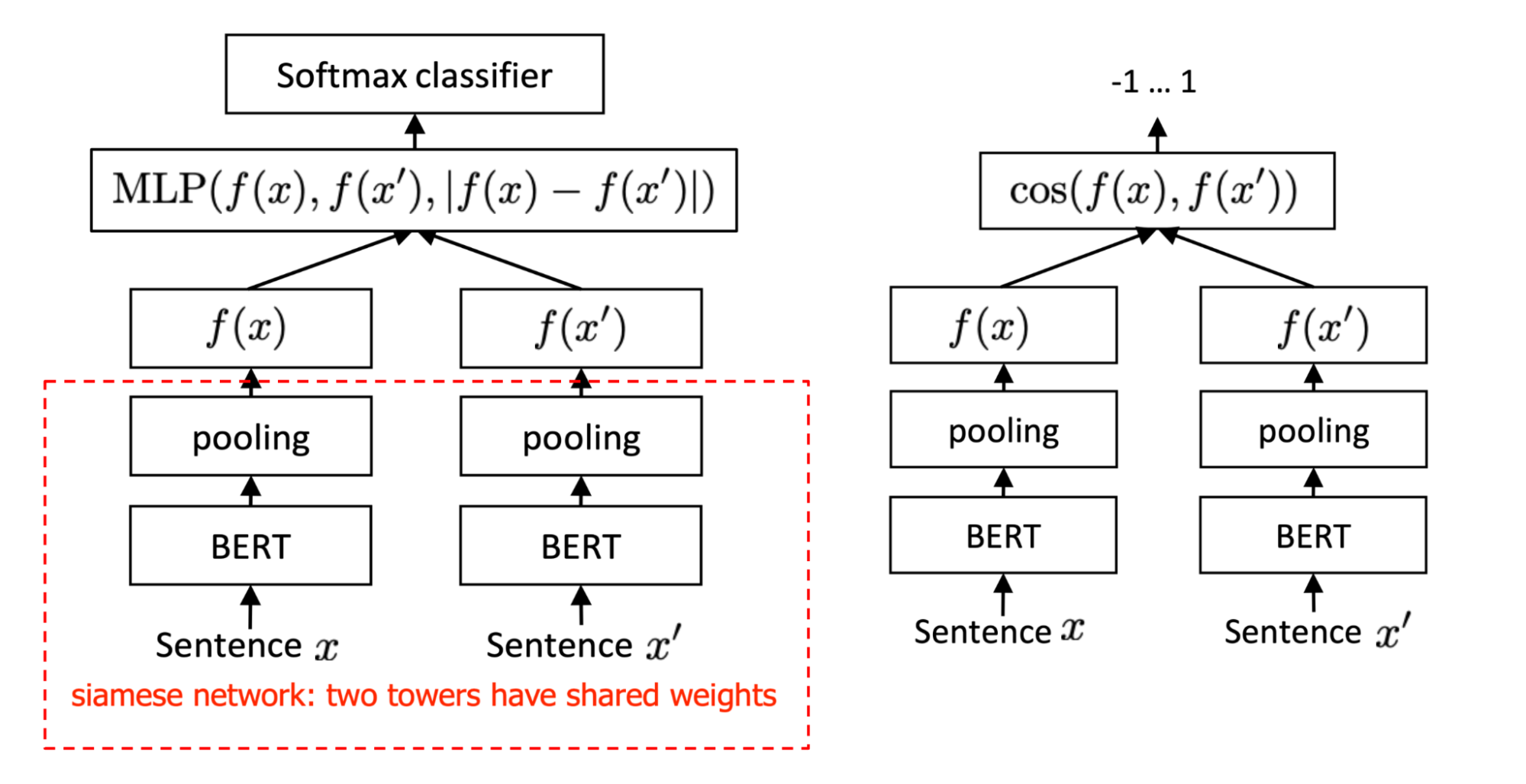Fig. 24. Illustration of Sentence-BERT training framework with softmax classification head and regression head. (Image source: Reimers & Gurevych, 2019)

The SentEval library (Conneau and Kiela, 2018) is commonly used for evaluating the quality of learned sentence embedding. SBERT outperformed other baselines at that time (Aug 2019) on 5 out of 7 tasks.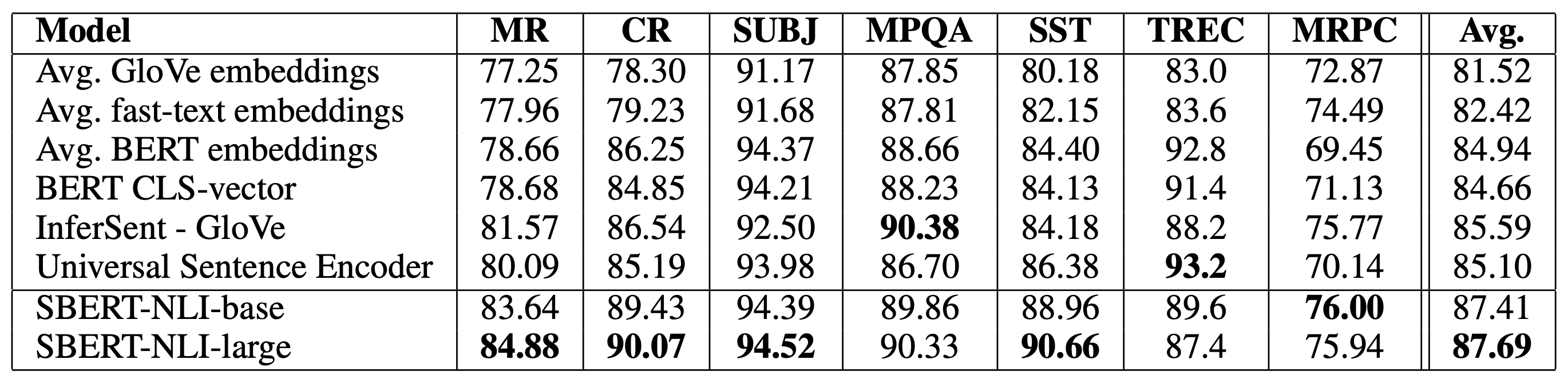Fig. 25. The performance of Sentence-BERT on the SentEval benchmark. (Image source: Reimers & Gurevych, 2019)

#### BERT-flow

The embedding representation space is deemed isotropic if embeddings are uniformly distributed on each dimension; otherwise, it is anisotropic. Li et al, (2020) showed that a pre-trained BERT learns a non-smooth anisotropic semantic space of sentence embeddings and thus leads to poor performance for text similarity tasks without fine-tuning. Empirically, they observed two issues with BERT sentence embedding: Word frequency biases the embedding space. High-frequency words are close to the origin, but low-frequency ones are far away from the origin. Low-frequency words scatter sparsely. The embeddings of low-frequency words tend to be farther to their $$k$$-NN neighbors, while the embeddings of high-frequency words concentrate more densely.

BERT-flow (Li et al, 2020; code) was proposed to transform the embedding to a smooth and isotropic Gaussian distribution via normalizing flows.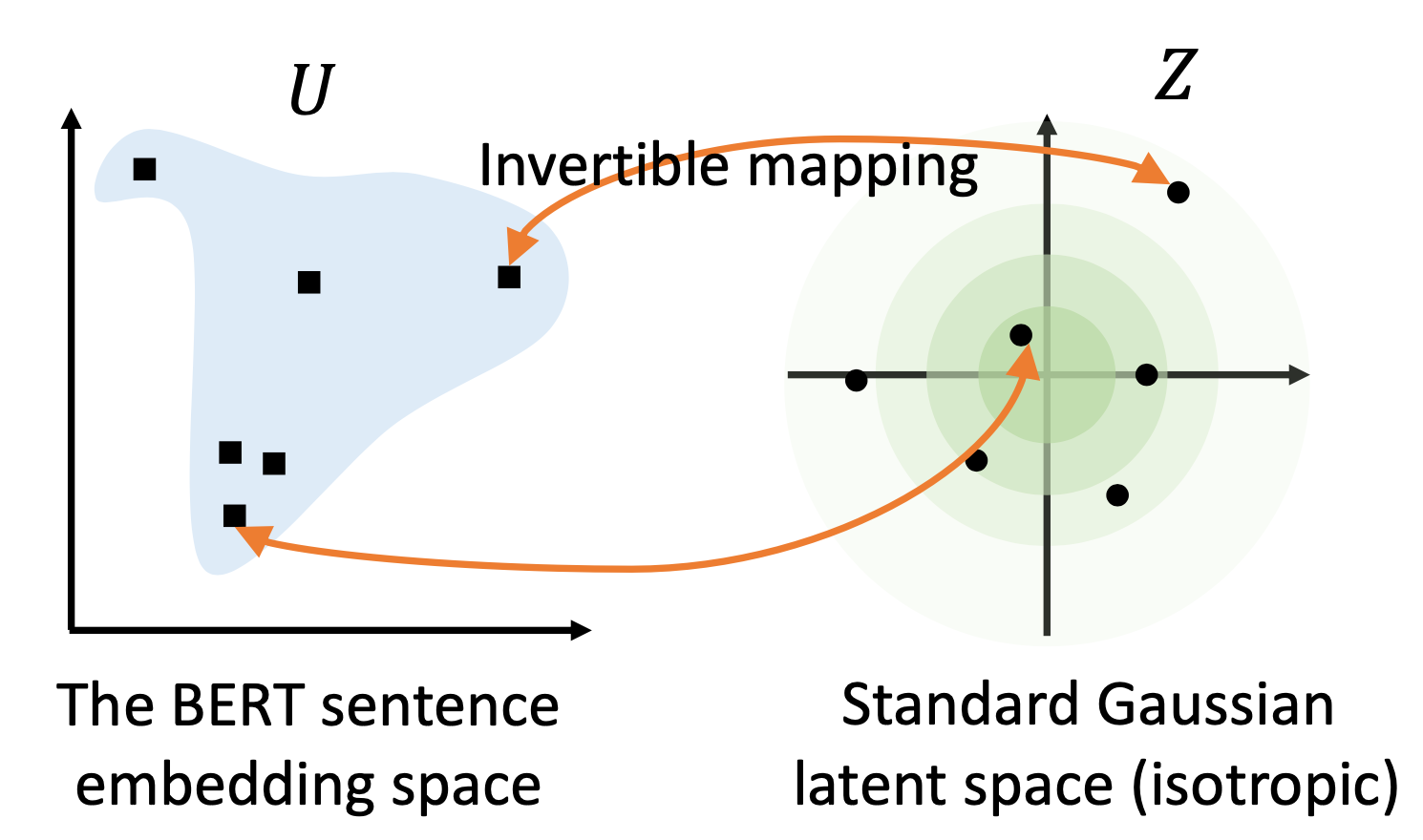Fig. 26. Illustration of the flow-based calibration over the original sentence embedding space in BERT-flow. (Image source: Li et al, 2020)

Let $$\mathcal{U}$$ be the observed BERT sentence embedding space and $$\mathcal{Z}$$ be the desired latent space which is a standard Gaussian. Thus, $$p_\mathcal{Z}$$ is a Gaussian density function and $$f_\phi: \mathcal{Z}\to\mathcal{U}$$ is an invertible transformation:

$\mathbf{z}\sim p_\mathcal{Z}(\mathbf{z}) \quad \mathbf{u}=f_\phi(\mathbf{z}) \quad \mathbf{z}=f^{-1}_\phi(\mathbf{u})$

A flow-based generative model learns the invertible mapping function by maximizing the likelihood of $$\mathcal{U}$$’s marginal:

$\max_\phi\mathbb{E}_{\mathbf{u}=\text{BERT}(s), s\sim\mathcal{D}} \Big[ \log p_\mathcal{Z}(f^{-1}_\phi(\mathbf{u})) + \log\big\vert\det\frac{\partial f^{-1}_\phi(\mathbf{u})}{\partial\mathbf{u}}\big\vert \Big]$

where $$s$$ is a sentence sampled from the text corpus $$\mathcal{D}$$. Only the flow parameters $$\phi$$ are optimized while parameters in the pretrained BERT stay unchanged.

BERT-flow was shown to improve the performance on most STS tasks either with or without supervision from NLI datasets. Because learning normalizing flows for calibration does not require labels, it can utilize the entire dataset including validation and test sets.

#### Whitening Operation

Su et al. (2021) applied whitening operation to improve the isotropy of the learned representation and also to reduce the dimensionality of sentence embedding.

They transform the mean value of the sentence vectors to 0 and the covariance matrix to the identity matrix. Given a set of samples $$\{\mathbf{x}_i\}_{i=1}^N$$, let $$\tilde{\mathbf{x}}_i$$ and $$\tilde{\Sigma}$$ be the transformed samples and corresponding covariance matrix:

\begin{aligned} \mu &= \frac{1}{N}\sum_{i=1}^N \mathbf{x}_i \quad \Sigma = \frac{1}{N}\sum_{i=1}^N (\mathbf{x}_i - \mu)^\top (\mathbf{x}_i - \mu) \\ \tilde{\mathbf{x}}_i &= (\mathbf{x}_i - \mu)W \quad \tilde{\Sigma} = W^\top\Sigma W = I \text{ thus } \Sigma = (W^{-1})^\top W^{-1} \end{aligned}

If we get SVD decomposition of $$\Sigma = U\Lambda U^\top$$, we will have $$W^{-1}=\sqrt{\Lambda} U^\top$$ and $$W=U\sqrt{\Lambda^{-1}}$$. Note that within SVD, $$U$$ is an orthogonal matrix with column vectors as eigenvectors and $$\Lambda$$ is a diagonal matrix with all positive elements as sorted eigenvalues.

A dimensionality reduction strategy can be applied by only taking the first $$k$$ columns of $$W$$, named Whitening-$$k$$.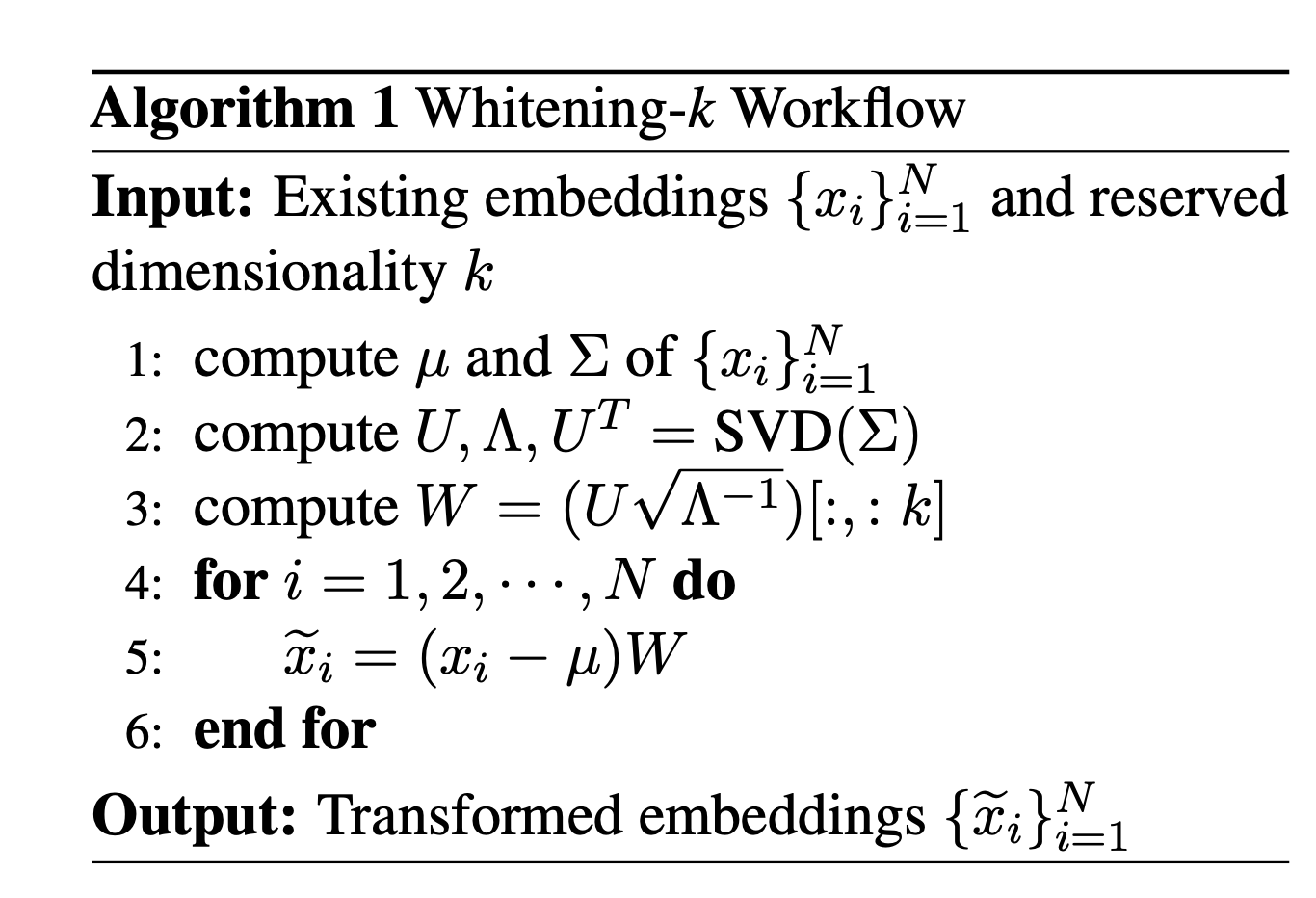Fig. 27. Pseudo code of the whitening-$$k$$ operation. (Image source: Su et al. 2021)

Whitening operations were shown to outperform BERT-flow and achieve SOTA with 256 sentence dimensionality on many STS benchmarks, either with or without NLI supervision.

### Unsupervised Sentence Embedding Learning

#### Context Prediction

Quick-Thought (QT) vectors (Logeswaran & Lee, 2018) formulate sentence representation learning as a classification problem: Given a sentence and its context, a classifier distinguishes context sentences from other contrastive sentences based on their vector representations (“cloze test”). Such a formulation removes the softmax output layer which causes training slowdown.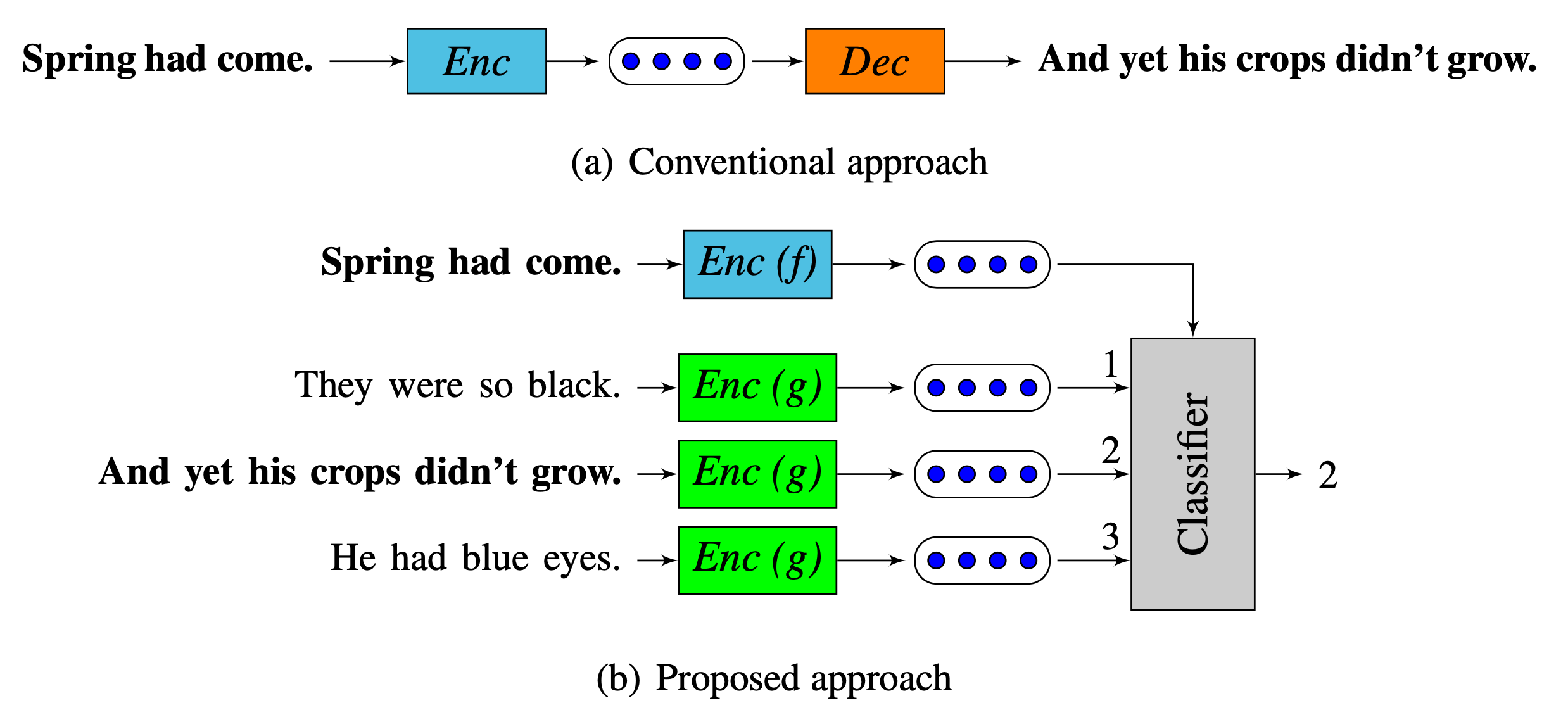Fig. 28. Illustration of how Quick-Thought sentence embedding vectors are learned. (Image source: Logeswaran & Lee, 2018)

Let $$f(.)$$ and $$g(.)$$ be two functions that encode a sentence $$s$$ into a fixed-length vector. Let $$C(s)$$ be the set of sentences in the context of $$s$$ and $$S(s)$$ be the set of candidate sentences including only one sentence $$s_c \in C(s)$$ and many other non-context negative sentences. Quick Thoughts model learns to optimize the probability of predicting the only true context sentence $$s_c \in S(s)$$. It is essentially NCE loss when considering the sentence $$(s, s_c)$$ as the positive pairs while other pairs $$(s, s')$$ where $$s' \in S(s), s'\neq s_c$$ as negatives.

$\mathcal{L}_\text{QT} = - \sum_{s \in \mathcal{D}} \sum_{s_c \in C(s)} \log p(s_c \vert s, S(s)) = - \sum_{s \in \mathcal{D}} \sum_{s_c \in C(s)}\frac{\exp(f(s)^\top g(s_c))}{\sum_{s'\in S(s)} \exp(f(s)^\top g(s'))}$

#### Mutual Information Maximization

IS-BERT (Info-Sentence BERT) (Zhang et al. 2020; code) adopts a self-supervised learning objective based on mutual information maximization to learn good sentence embeddings in the unsupervised manners.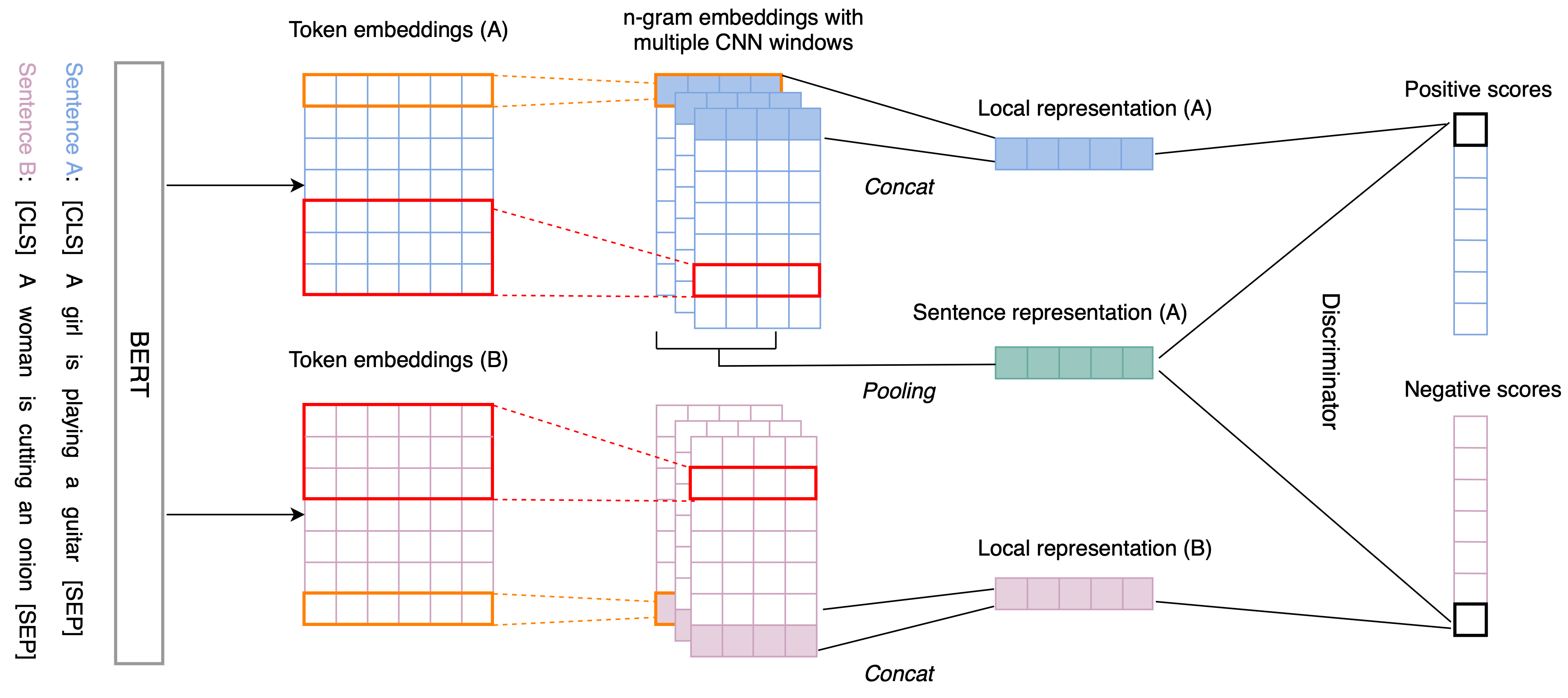Fig. 29. Illustration of Info-Sentence BERT. (Image source: Zhang et al. 2020)

IS-BERT works as follows:

1. Use BERT to encode an input sentence $$s$$ to a token embedding of length $$l$$, $$\mathbf{h}_{1:l}$$.

2. Then apply 1-D conv net with different kernel sizes (e.g. 1, 3, 5) to process the token embedding sequence to capture the n-gram local contextual dependencies: $$\mathbf{c}_i = \text{ReLU}(\mathbf{w} \cdot \mathbf{h}_{i:i+k-1} + \mathbf{b})$$. The output sequences are padded to stay the same sizes of the inputs.
3. The final local representation of the $$i$$-th token $$\mathcal{F}_\theta^{(i)} (\mathbf{x})$$ is the concatenation of representations of different kernel sizes.
4. The global sentence representation $$\mathcal{E}_\theta(\mathbf{x})$$ is computed by applying a mean-over-time pooling layer on the token representations $$\mathcal{F}_\theta(\mathbf{x}) = \{\mathcal{F}_\theta^{(i)} (\mathbf{x}) \in \mathbb{R}^d\}_{i=1}^l$$.

Since the mutual information estimation is generally intractable for continuous and high-dimensional random variables, IS-BERT relies on the Jensen-Shannon estimator (Nowozin et al., 2016, Hjelm et al., 2019) to maximize the mutual information between $$\mathcal{E}_\theta(\mathbf{x})$$ and $$\mathcal{F}_\theta^{(i)} (\mathbf{x})$$.

$I^\text{JSD}_\omega(\mathcal{F}_\theta^{(i)} (\mathbf{x}); \mathcal{E}_\theta(\mathbf{x})) = \mathbb{E}_{\mathbf{x}\sim P} [-\text{sp}(-T_\omega(\mathcal{F}_\theta^{(i)} (\mathbf{x}); \mathcal{E}_\theta(\mathbf{x})))] \\ - \mathbb{E}_{\mathbf{x}\sim P, \mathbf{x}' \sim\tilde{P}} [\text{sp}(T_\omega(\mathcal{F}_\theta^{(i)} (\mathbf{x}'); \mathcal{E}_\theta(\mathbf{x})))]$

where $$T_\omega: \mathcal{F}\times\mathcal{E} \to \mathbb{R}$$ is a learnable network with parameters $$\omega$$, generating discriminator scores. The negative sample $$\mathbf{x}'$$ is sampled from the distribution $$\tilde{P}=P$$. And $$\text{sp}(x)=\log(1+e^x)$$ is the softplus activation function.

The unsupervised numbers on SentEval with IS-BERT outperforms most of the unsupervised baselines (Sep 2020), but unsurprisingly weaker than supervised runs. When using labelled NLI datasets, IS-BERT produces results comparable with SBERT (See Fig. 25 & 30).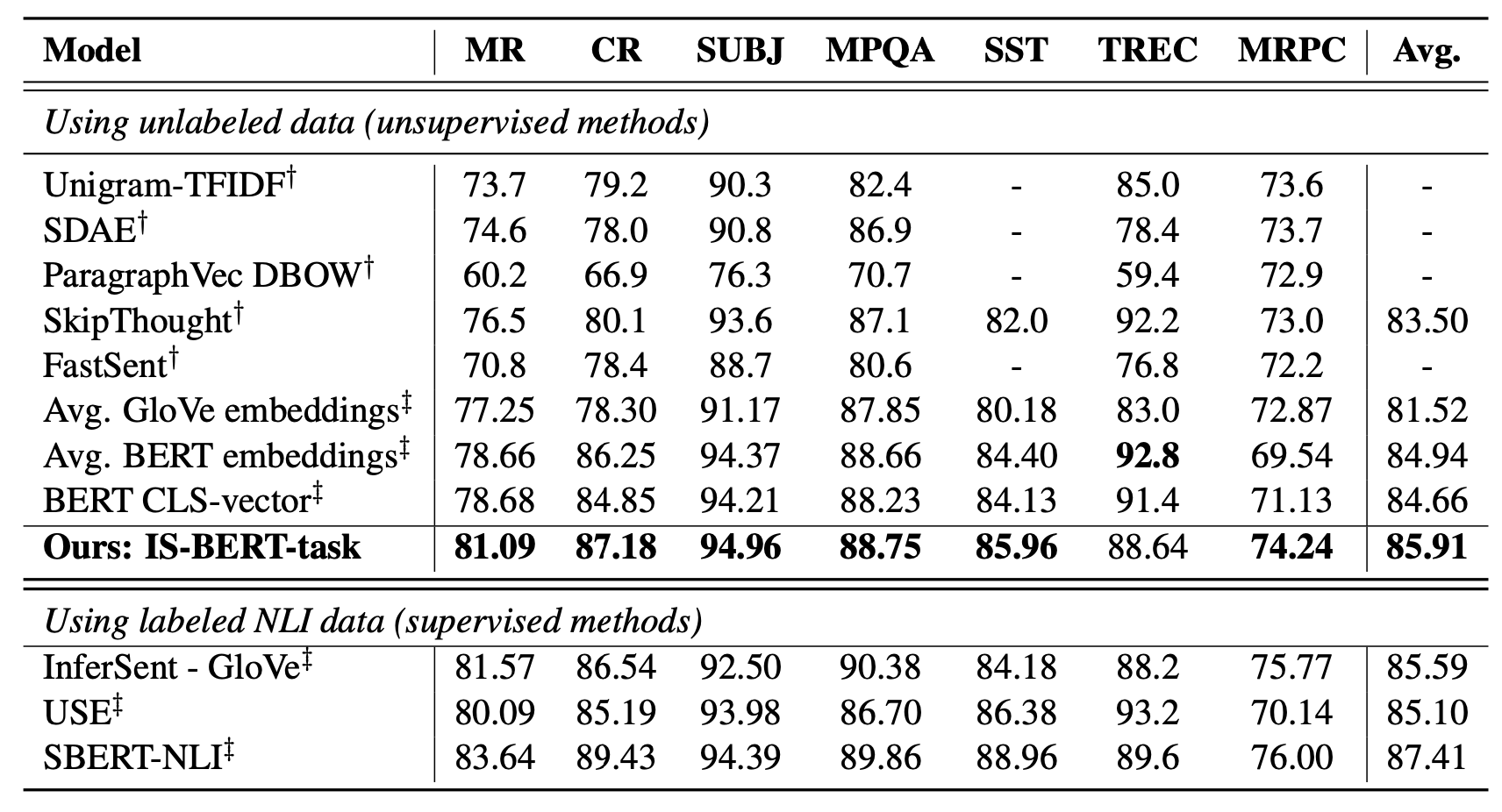Fig. 30. The performance of IS-BERT on the SentEval benchmark. (Image source: Zhang et al. 2020)

Cited as:

@article{weng2021contrastive,
title   = "Contrastive Representation Learning",
author  = "Weng, Lilian",
journal = "lilianweng.github.io/lil-log",
year    = "2021",
url     = "https://lilianweng.github.io/lil-log/2021/05/31/contrastive-representation-learning.html"
}


 Sumit Chopra, Raia Hadsell and Yann LeCun. “Learning a similarity metric discriminatively, with application to face verification.” CVPR 2005.

 Florian Schroff, Dmitry Kalenichenko and James Philbin. “FaceNet: A Unified Embedding for Face Recognition and Clustering.” CVPR 2015.

 Hyun Oh Song et al. “Deep Metric Learning via Lifted Structured Feature Embedding.” CVPR 2016. [code]

 Ruslan Salakhutdinov and Geoff Hinton. “Learning a Nonlinear Embedding by Preserving Class Neighbourhood Structure” AISTATS 2007.

 Michael Gutmann and Aapo Hyvärinen. “Noise-contrastive estimation: A new estimation principle for unnormalized statistical models.” AISTATS 2010.

 Kihyuk Sohn et al. “Improved Deep Metric Learning with Multi-class N-pair Loss Objective” NIPS 2016.

 Nicholas Frosst, Nicolas Papernot and Geoffrey Hinton. “Analyzing and Improving Representations with the Soft Nearest Neighbor Loss.” ICML 2019

 Tongzhou Wang and Phillip Isola. “Understanding Contrastive Representation Learning through Alignment and Uniformity on the Hypersphere.” ICML 2020. [code]

 Zhirong Wu et al. “Unsupervised feature learning via non-parametric instance-level discrimination.” CVPR 2018.

 Ekin D. Cubuk et al. “AutoAugment: Learning augmentation policies from data.” arXiv preprint arXiv:1805.09501 (2018).

 Daniel Ho et al. “Population Based Augmentation: Efficient Learning of Augmentation Policy Schedules.” ICML 2019.

 Ekin D. Cubuk & Barret Zoph et al. “RandAugment: Practical automated data augmentation with a reduced search space.” arXiv preprint arXiv:1909.13719 (2019).

 Hongyi Zhang et al. “mixup: Beyond Empirical Risk Minimization.” ICLR 2017.

 Sangdoo Yun et al. “CutMix: Regularization Strategy to Train Strong Classifiers with Localizable Features.” ICCV 2019.

 Yannis Kalantidis et al. “Mixing of Contrastive Hard Negatives” NeuriPS 2020.

 Ashish Jaiswal et al. “A Survey on Contrastive Self-Supervised Learning.” arXiv preprint arXiv:2011.00362 (2021)

 Jure Zbontar et al. “Barlow Twins: Self-Supervised Learning via Redundancy Reduction.” arXiv preprint arXiv:2103.03230 (2021) [code]

 Alec Radford, et al. “Learning Transferable Visual Models From Natural Language Supervision” arXiv preprint arXiv:2103.00020 (2021)

 Mathilde Caron et al. “Unsupervised Learning of Visual Features by Contrasting Cluster Assignments (SwAV).” NeuriPS 2020.

 Mathilde Caron et al. “Deep Clustering for Unsupervised Learning of Visual Features.” ECCV 2018.

 Prannay Khosla et al. “Supervised Contrastive Learning.” NeurIPS 2020.

 Aaron van den Oord, Yazhe Li & Oriol Vinyals. “Representation Learning with Contrastive Predictive Coding” arXiv preprint arXiv:1807.03748 (2018).

 Jason Wei and Kai Zou. “EDA: Easy data augmentation techniques for boosting performance on text classification tasks.” EMNLP-IJCNLP 2019.

 Sosuke Kobayashi. “Contextual Augmentation: Data Augmentation by Words with Paradigmatic Relations.” NAACL 2018

 Hongchao Fang et al. “CERT: Contrastive self-supervised learning for language understanding.” arXiv preprint arXiv:2005.12766 (2020).

 Dinghan Shen et al. “A Simple but Tough-to-Beat Data Augmentation Approach for Natural Language Understanding and Generation.” arXiv preprint arXiv:2009.13818 (2020) [code]

 Tianyu Gao et al. “SimCSE: Simple Contrastive Learning of Sentence Embeddings.” arXiv preprint arXiv:2104.08821 (2020). [code]

 Nils Reimers and Iryna Gurevych. “Sentence-BERT: Sentence embeddings using Siamese BERT-networks.” EMNLP 2019.

 Jianlin Su et al. “Whitening sentence representations for better semantics and faster retrieval.” arXiv preprint arXiv:2103.15316 (2021). [code]

 Yan Zhang et al. “An unsupervised sentence embedding method by mutual information maximization.” EMNLP 2020. [code]

 Bohan Li et al. “On the sentence embeddings from pre-trained language models.” EMNLP 2020.

 Lajanugen Logeswaran and Honglak Lee. “An efficient framework for learning sentence representations.” ICLR 2018.

 Joshua Robinson, et al. “Contrastive Learning with Hard Negative Samples.” ICLR 2021.

 Ching-Yao Chuang et al. “Debiased Contrastive Learning.” NeuriPS 2020.